### Afternoondragon Step04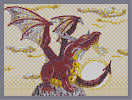Hover over the thumbnail for a full-size version.

Author Dragon_Moon author:dragon_moon bitesized featured n-art rated 2006-03-17 2008-05-25 5 by 1265 people. \$Afternoondragon Step04#Dragon_Moon#none#00000000000000000000000000000000000000000000000000000000000000000000000000000000000000000000000000000000000000000000000000000000000000000000000000000000000000000000000000000000000000000000000000000000000000000000000000000000000000000000000000000000000000000000000000000000000000000000000000000000000000000000000000000000000000000000000000000000000000000000000000000000000000000000000000000000000000000000000000000000000000000000000000000000000000000000000000000000000000000000000000000000000000000000000000000000000000000000000000000000000000000000000000000000000000000000000000000000000000000000000000000000000000000000000000000000000000000000000000000000000000000000000000000000000000000000000000000000000000000|5^423,170!5^465,118!5^307,561!5^642,436!5^598,206!12^660,414!12^605,203!12^601,203!12^595,203!12^589,203!12^578,205!12^572,208!12^585,205!12^575,207!12^568,211!12^566,213!12^562,216!12^558,221!12^553,225!12^550,228!12^546,232!12^542,235!12^535,240!12^525,244!12^520,247!12^514,250!12^507,254!12^503,256!12^501,261!12^495,266!12^492,266!12^498,265!12^540,237!12^522,246!12^531,242!12^581,204!12^511,252!12^517,249!12^604,200!12^600,197!12^594,194!12^590,194!12^587,193!12^582,192!12^575,192!12^569,192!12^553,201!12^549,204!12^544,208!12^538,210!12^532,210!12^526,210!12^523,210!12^517,210!12^511,211!12^504,212!12^499,213!12^494,217!12^490,219!12^485,223!12^483,226!12^477,232!12^479,228!12^475,233!12^472,236!12^468,238!12^461,240!12^454,242!12^457,241!12^465,239!12^500,230!12^504,230!12^504,228!12^508,228!12^512,228!12^525,220!12^522,223!12^520,227!12^520,223!12^516,224!12^512,224!12^511,224!12^508,224!12^519,225!12^504,226!0^505,227!0^510,226!0^515,225!12^594,210!12^594,212!12^593,216!12^593,220!12^593,223!12^592,228!12^591,230!12^590,233!12^588,236!12^587,240!12^586,245!12^583,247!12^581,250!12^578,254!12^577,258!12^575,261!12^572,262!12^566,264!12^559,266!12^553,266!12^547,270!12^544,275!12^540,280!12^537,284!12^532,289!12^529,294!12^526,298!12^521,301!12^471,282!12^515,300!12^509,298!12^505,300!12^500,297!12^495,295!12^488,295!12^487,290!12^482,287!12^475,287!12^478,287!12^472,285!12^492,296!12^500,299!7^593,253,2!7^570,261,2!7^581,259,2!7^573,275,2!7^596,277,2!7^576,282,1!7^581,272,1!7^588,298,1!7^600,292,1!7^613,313,1!7^625,305,1!7^643,304,1!7^627,287,1!7^597,280,1!7^583,261,1!7^553,267,1!7^572,241,1!2^529,202,-0.707106781186547,-0.707106781186547!2^538,192,-1,0!2^535,205,-0.707106781186547,-0.707106781186547!2^514,309,-0.707106781186547,-0.707106781186547!2^507,319,-0.707106781186547,-0.707106781186547!2^508,308,-0.707106781186547,-0.707106781186547!2^501,318,-0.707106781186547,-0.707106781186547!2^498,329,-0.707106781186547,-0.707106781186547!2^492,336,-1,0!2^492,333,-0.707106781186547,-0.707106781186547!2^488,346,-0.707106781186547,-0.707106781186547!2^487,352,-1,0!2^524,207,0,-1!12^449,242!12^444,243!12^441,245!12^438,245!12^434,247!12^430,248!12^429,248!12^427,251!12^424,252!12^422,252!12^420,254!12^417,256!12^414,258!12^411,258!12^408,260!12^407,261!12^405,263!12^403,264!12^401,266!12^399,267!12^396,270!12^395,272!12^392,275!12^390,278!12^387,282!12^384,285!12^382,288!12^380,293!12^378,296!12^375,302!12^376,301!12^375,308!12^375,312!12^375,319!12^375,306!12^376,317!12^376,324!12^376,328!12^376,333!12^376,337!12^377,341!12^378,341!12^378,345!12^378,348!12^378,352!12^378,357!12^377,361!12^376,363!12^375,366!2^458,239,0,-1!2^446,237,0,-1!2^433,234,0,-1!2^423,230,0,-1!2^411,228,0.707106781186547,-0.707106781186547!2^403,223,0,-1!2^392,222,0.707106781186547,-0.707106781186547!2^387,218,0,-1!2^378,218,0.707106781186547,-0.707106781186547!2^370,214,0,-1!2^365,210,0.707106781186547,-0.707106781186547!2^357,204,0.707106781186547,-0.707106781186547!2^355,196,0.707106781186547,-0.707106781186547!2^364,217,0.707106781186547,-0.707106781186547!2^373,222,0.707106781186547,-0.707106781186547!2^388,226,0.707106781186547,-0.707106781186547!2^387,229,0,-1!2^403,230,0.707106781186547,-0.707106781186547!2^399,230,0,-1!2^417,239,0.707106781186547,-0.707106781186547!2^428,240,0,-1!2^431,242,-0.707106781186547,-0.707106781186547!2^440,240,0,-1!2^420,235,0,-1!2^394,206,0.707106781186547,-0.707106781186547!2^408,214,0.707106781186547,-0.707106781186547!2^403,215,0.707106781186547,-0.707106781186547!2^416,220,0.707106781186547,-0.707106781186547!2^428,223,0,-1!2^436,228,0.707106781186547,-0.707106781186547!2^450,234,0,-1!2^393,200,0.707106781186547,-0.707106781186547!2^496,308,-0.707106781186547,-0.707106781186547!2^490,304,-0.707106781186547,-0.707106781186547!2^483,316,-0.707106781186547,-0.707106781186547!2^488,313,-0.707106781186547,-0.707106781186547!2^477,323,-0.707106781186547,-0.707106781186547!2^476,330,-1,0!2^472,338,-0.707106781186547,-0.707106781186547!2^468,345,-1,0!2^472,334,-1,0!2^483,298,-0.707106781186547,-0.707106781186547!2^474,304,-0.707106781186547,-0.707106781186547!2^465,309,-1,0!2^462,317,-0.707106781186547,-0.707106781186547!2^457,323,-1,0!2^455,334,-0.707106781186547,-0.707106781186547!2^454,328,-1,0!2^450,337,-1,0!2^459,311,-0.707106781186547,-0.707106781186547!2^469,303,-0.707106781186547,-0.707106781186547!2^478,297,-0.707106781186547,-0.707106781186547!2^467,289,0,-1!2^457,291,0,-1!2^451,295,0,-1!2^444,301,-0.707106781186547,-0.707106781186547!2^438,309,0,-1!2^434,313,-1,0!2^438,312,-0.707106781186547,-0.707106781186547!2^446,301,-0.707106781186547,-0.707106781186547!2^455,298,-0.707106781186547,-0.707106781186547!2^462,293,0,-1!2^471,292,0,-1!12^558,197!12^564,194!12^578,192!12^598,196!12^562,193!0^480,306!0^475,311!0^470,315!0^465,320!0^462,326!0^460,332!0^457,340!0^458,346!0^460,352!0^462,359!0^467,373!0^465,367!0^464,363!0^458,336!12^472,278!12^492,293!12^497,290!12^507,297!12^509,293!12^479,282!0^471,380!0^470,378!0^473,387!0^470,391!0^471,396!0^473,402!0^473,408!0^471,415!0^467,428!0^466,435!0^465,439!0^464,445!0^460,445!0^465,392!0^462,395!0^456,396!0^451,395!0^446,392!0^457,352!0^452,353!0^447,353!0^443,352!0^440,346!0^438,341!0^441,390!0^437,387!0^435,383!0^435,379!0^459,446!0^455,445!0^452,445!0^439,441!0^433,435!0^431,431!0^428,424!0^426,419!0^437,439!0^428,415!12^420,441!12^419,443!12^417,448!12^417,453!12^416,458!12^412,465!12^408,471!12^408,473!12^408,476!12^409,470!12^401,416!12^396,427!12^396,432!12^396,437!12^396,443!12^396,447!12^399,457!12^409,481!12^410,485!12^412,490!12^414,497!12^416,504!12^420,509!12^416,512!12^423,513!12^427,516!12^430,519!12^452,544!12^451,546!12^441,549!12^442,524!12^447,524!12^453,526!12^459,530!12^462,534!12^465,539!12^426,558!12^423,553!12^423,549!12^425,546!12^420,549!12^417,549!12^413,546!12^376,517!12^373,514!12^372,512!12^375,499!12^375,492!12^373,509!12^374,505!12^376,497!12^374,488!12^374,484!12^369,478!12^368,475!12^365,472!12^362,465!12^360,460!12^360,456!12^361,446!0^458,451!0^458,458!0^457,464!0^454,470!0^450,475!0^445,481!0^441,487!0^435,491!0^430,488!0^424,485!0^419,481!0^415,479!0^413,476!0^430,493!0^428,498!0^424,504!0^425,505!0^427,501!12^361,450!12^398,422!12^399,452!12^374,368!12^372,368!12^371,370!12^370,370!12^350,375!12^346,373!12^343,372!12^338,370!12^336,370!12^331,370!12^328,369!12^325,369!12^319,369!12^314,369!12^309,369!12^296,372!12^293,374!12^269,388!12^265,391!12^263,393!12^252,415!12^251,420!12^250,429!12^305,369!12^300,370!12^289,376!12^286,378!12^282,380!12^277,383!12^272,385!12^261,396!12^258,400!12^256,404!12^254,409!12^250,424!12^248,432!12^357,378!12^372,359!12^368,355!12^366,354!12^363,351!12^357,349!12^355,348!12^350,344!12^346,341!12^343,339!12^339,335!12^336,332!12^332,329!12^329,325!12^326,322!12^323,318!12^319,312!12^315,308!12^311,303!12^308,298!12^304,293!12^302,289!12^300,287!12^294,281!12^290,279!12^286,277!12^282,277!12^280,277!12^296,284!12^282,274!12^282,274!12^285,270!12^289,267!12^292,262!12^293,261!12^299,221!12^297,214!12^296,213!12^294,208!12^295,257!12^298,253!12^300,247!12^300,242!12^301,239!12^301,237!12^301,234!12^301,232!12^300,229!12^300,226!12^299,224!12^297,216!12^294,205!12^294,203!12^293,199!12^293,196!12^293,193!12^293,190!12^293,188!12^293,185!12^293,181!12^293,176!12^294,173!12^294,170!12^294,166!12^294,161!12^294,157!12^299,151!12^295,154!12^305,148!12^310,150!12^316,147!12^317,143!12^312,131!12^308,131!12^304,131!12^299,132!12^291,132!12^294,134!12^286,128!12^270,117!12^251,105!12^230,91!12^203,77!12^281,125!12^278,122!12^275,120!12^266,113!12^262,111!12^255,108!12^257,109!12^248,103!12^246,102!12^243,100!12^240,97!12^238,96!12^236,95!12^234,93!12^231,91!12^228,90!12^224,87!12^219,84!12^215,82!12^212,80!12^206,77!12^203,76!12^200,74!12^198,73!12^195,71!12^192,70!12^190,68!12^187,67!12^181,65!12^176,62!12^171,60!12^166,58!12^161,56!12^157,55!12^154,54!12^148,51!12^147,51!12^142,49!12^137,48!12^135,48!12^127,46!12^122,45!12^118,44!12^112,43!12^108,42!12^102,41!12^96,41!12^89,41!12^84,41!12^79,42!12^69,43!12^67,43!12^63,43!12^55,44!12^51,45!12^45,45!12^58,44!12^91,41!12^130,47!12^73,42!12^151,53!12^59,148!12^297,370!12^299,367!12^299,363!12^299,357!12^296,352!12^292,349!12^290,346!12^287,342!12^132,235!12^132,232!12^132,231!12^133,226!12^134,221!12^135,215!12^56,150!12^54,151!12^53,151!12^52,152!12^53,151!12^58,145!12^60,144!12^32,56!12^34,52!12^36,50!12^42,47!12^45,45!12^28,55!12^61,141!12^48,154!12^295,349!12^289,344!12^285,339!12^282,336!12^278,331!12^276,326!12^274,323!12^271,320!12^268,315!12^266,311!12^264,306!12^258,293!12^257,291!12^254,284!12^250,282!12^249,282!12^245,279!12^245,276!12^246,271!12^250,268!12^251,263!12^252,259!12^255,253!12^255,252!12^255,245!12^256,240!12^258,230!12^259,225!12^260,220!12^260,216!12^256,247!12^255,288!12^260,213!12^260,211!12^262,201!12^264,196!12^265,192!12^261,208!12^267,187!12^267,186!12^268,179!12^268,173!12^270,166!12^272,162!12^275,156!12^278,153!12^280,148!12^279,149!12^272,149!12^266,149!12^264,149!12^258,149!12^253,149!12^252,150!12^247,150!12^243,150!12^237,150!12^233,150!12^226,151!12^220,153!12^210,157!12^206,157!12^201,160!12^197,161!12^187,167!12^182,169!12^178,172!12^174,175!12^170,179!12^157,190!12^152,195!12^148,198!12^143,205!12^138,213!12^135,218!12^125,241!12^127,238!12^146,201!12^141,209!12^153,192!12^160,187!12^165,183!12^191,164!12^215,154!12^217,151!12^219,151!12^220,151!12^224,148!12^225,147!12^230,146!12^232,146!12^235,145!12^237,145!12^240,144!12^242,144!12^245,143!12^249,143!12^253,143!12^256,143!12^259,142!12^263,142!12^265,142!12^267,142!12^270,142!12^273,142!12^276,142!12^277,142!12^219,147!12^217,149!12^213,152!12^212,152!12^208,155!12^208,156!12^207,154!12^204,155!12^200,156!12^195,159!12^188,163!12^193,162!12^184,168!12^274,147!12^270,139!12^264,137!12^259,135!12^257,133!12^253,130!12^249,128!12^246,126!12^243,125!12^237,122!12^232,120!12^228,119!12^221,118!12^216,117!12^209,116!12^202,116!12^123,117!12^116,119!12^108,120!12^102,122!12^96,125!12^93,126!12^84,132!12^81,134!12^79,135!12^74,139!12^69,141!12^66,142!12^64,142!12^61,145!12^71,141!12^98,123!12^104,122!12^104,122!12^112,120!12^128,118!12^119,118!12^200,116!12^87,128!12^90,128!12^272,136!12^269,136!12^265,132!12^263,130!12^261,130!12^258,127!12^255,124!12^251,123!12^249,123!12^246,123!12^245,123!12^243,122!12^240,119!12^237,118!12^234,117!12^231,116!12^225,115!12^223,114!12^219,114!12^215,114!12^210,114!12^206,114!12^129,116!12^225,119!12^204,114!12^202,114!12^198,114!12^242,122!12^133,116!12^140,115!12^141,114!12^145,114!12^148,114!12^150,113!12^154,113!12^158,113!12^161,113!12^166,113!12^172,113!12^176,113!12^185,113!12^193,113!12^198,114!12^192,113!12^184,112!12^181,113!12^187,115!12^191,115!12^197,116!12^194,116!12^181,115!12^180,114!12^177,114!12^173,114!12^168,114!12^164,114!12^159,114!12^137,116!12^76,137!12^37,51!12^281,131!12^279,129!12^271,122!12^268,121!12^267,120!12^261,115!12^283,135!12^277,131!12^274,129!12^270,128!12^266,125!12^263,120!12^262,119!12^260,117!12^258,116!12^256,115!12^254,113!12^252,112!12^251,111!12^249,110!12^246,108!12^246,106!12^244,105!12^243,104!12^241,102!12^239,101!12^236,100!12^234,98!12^232,97!12^230,96!12^228,94!12^225,92!12^223,90!12^219,88!12^217,87!12^215,85!12^212,83!12^210,82!12^207,80!12^204,78!12^200,77!12^197,75!12^192,72!12^187,70!12^182,68!12^181,67!12^178,67!12^175,64!12^172,64!12^170,61!12^168,60!12^166,59!12^162,58!12^157,57!12^152,55!12^150,54!12^146,53!12^141,50!12^142,52!12^136,49!12^274,124!12^286,133!12^278,137!12^274,135!12^271,133!12^265,128!12^261,124!12^270,384!12^440,553!12^372,482!12^363,471!12^418,445!2^432,554,1,0!2^435,562,1,0!2^433,567,0.707106781186547,0.707106781186547!2^427,560,1,0!2^432,561,1,0!2^431,557,1,0!2^429,568,0.707106781186547,0.707106781186547!2^445,549,0.707106781186547,-0.707106781186547!2^448,556,0.707106781186547,-0.707106781186547!2^452,554,0.707106781186547,-0.707106781186547!2^455,560,1,0!2^453,560,1,0!2^460,546,0.707106781186547,-0.707106781186547!2^462,542,0.707106781186547,-0.707106781186547!2^466,549,1,0!2^466,550,1,0!2^467,552,1,0!2^316,137,-0.707106781186547,-0.707106781186547!2^314,141,-0.707106781186547,-0.707106781186547!2^310,139,0,-1!2^311,140,0,-1!2^310,138,-0.707106781186547,-0.707106781186547!2^318,132,0,-1!2^327,133,0,-1!2^334,134,0.707106781186547,-0.707106781186547!2^320,136,0,-1!2^333,134,0.707106781186547,-0.707106781186547!2^331,134,0,-1!12^495,292!12^509,296!12^472,280!12^287,379!12^293,378!12^295,375!12^295,379!12^305,466!12^305,466!12^304,468!12^304,469!12^302,470!12^301,472!12^299,476!12^298,478!12^297,480!12^295,481!12^294,484!12^292,485!12^290,485!12^288,486!12^285,489!12^283,491!12^282,492!12^278,493!12^275,493!12^249,440!12^249,446!12^248,435!12^250,451!12^251,453!12^251,454!12^251,456!12^251,457!12^253,461!12^253,464!12^254,467!12^255,470!12^255,472!12^256,477!12^257,479!12^258,482!12^260,486!12^260,487!12^263,488!12^265,490!12^266,491!12^267,493!12^269,493!12^273,495!12^288,490!12^288,489!12^249,439!12^275,494!12^262,491!12^295,491!12^295,491!12^295,495!12^296,499!12^298,503!12^299,508!12^301,510!12^301,511!12^304,516!12^305,522!12^307,523!12^264,496!12^263,500!12^263,505!12^262,507!12^261,510!12^261,511!12^260,515!12^259,517!12^258,519!12^257,523!12^255,525!12^255,528!12^255,529!12^308,528!12^308,528!12^266,535!12^264,532!12^261,530!12^259,530!12^256,530!12^253,532!12^252,538!12^252,543!12^254,545!12^255,550!12^255,553!12^267,539!12^269,541!12^269,544!12^269,547!12^269,552!12^273,536!12^273,535!12^275,532!12^275,530!12^278,527!12^280,526!12^283,523!12^285,522!12^289,525!12^290,531!12^291,534!12^291,539!12^291,544!12^291,549!12^291,552!12^288,555!12^288,556!12^287,560!12^271,555!12^274,560!12^267,556!12^298,534!12^301,532!12^302,531!12^304,530!12^307,530!12^313,532!12^313,538!12^313,542!12^313,546!12^311,549!12^310,552!12^309,552!12^309,554!12^296,540!12^297,544!12^296,548!12^296,558!12^295,554!12^412,547!12^373,517!12^371,513!12^371,506!12^423,552!12^422,511!12^418,511!12^418,507!12^415,500!12^413,493!12^411,490!12^408,484!12^405,477!12^453,525!12^452,525!12^444,523!12^438,522!12^434,522!12^410,547!12^409,547!12^407,546!12^404,546!12^402,546!12^402,546!12^398,544!12^395,539!12^393,536!12^391,533!12^389,529!12^387,527!12^382,523!12^380,522!12^376,520!2^385,536,-1,0!2^260,556,-1,0!2^263,561,-1,0!2^261,566,-1,0!2^278,555,-1,0!2^280,555,1,0!2^275,556,1,0!2^277,553,1,0!2^277,563,1,0!2^277,570,1,0!2^300,555,1,0!2^304,550,1,0!2^304,559,1,0!2^306,560,1,0!2^307,568,1,0!0^334,509!0^356,518!0^362,522!0^369,524!0^311,500!0^307,500!0^350,518!0^343,519!0^337,518!0^337,513!0^339,510!0^344,507!0^349,502!0^351,499!0^354,494!0^354,487!0^354,481!0^330,511!0^323,510!0^318,508!0^314,508!0^318,504!0^321,499!0^325,494!0^327,490!0^327,481!0^326,486!0^324,475!0^303,500!0^307,492!0^310,488!0^310,486!0^310,480!0^305,496!0^309,475!0^309,473!0^252,482!0^249,477!0^245,468!0^243,462!0^242,454!0^242,443!0^242,450!0^244,460!0^248,473!0^245,465!0^243,440!0^245,437!12^247,438!0^256,487!12^260,486!0^245,432!0^246,427!0^243,437!12^247,437!12^249,432!12^249,425!12^464,454!12^469,457!12^473,462!12^480,471!12^480,471!12^484,472!12^488,476!12^492,478!12^496,480!12^500,482!12^503,483!12^507,484!12^510,485!12^513,486!12^517,487!12^522,487!12^526,487!12^530,487!12^535,487!12^539,487!12^542,487!12^545,487!12^550,487!12^556,486!12^560,484!12^563,483!12^569,481!12^572,480!12^574,479!12^579,478!12^582,476!12^584,473!12^589,470!12^592,468!12^594,467!12^596,466!12^597,466!12^598,463!12^600,462!12^600,461!12^602,459!12^605,457!12^607,455!12^609,453!12^611,451!12^612,450!12^613,449!12^615,448!12^617,446!12^617,445!12^619,444!12^620,443!12^622,441!12^623,441!12^623,440!12^626,437!12^627,437!12^628,435!12^630,433!12^632,431!12^633,430!12^634,429!12^635,428!12^637,426!12^638,425!12^587,472!12^581,476!12^578,478!12^576,479!12^571,480!12^568,480!12^565,481!12^563,481!12^560,483!12^556,483!12^550,485!12^486,475!12^483,474!12^480,471!12^479,469!12^477,466!12^475,464!12^472,461!12^471,460!12^470,460!12^468,458!12^466,457!12^465,452!0^459,454!0^463,448!12^631,413!12^633,412!12^628,415!12^624,417!12^624,417!12^621,420!12^620,422!12^622,417!12^627,414!12^630,412!12^633,409!12^642,400!12^647,395!12^651,391!12^654,387!12^658,382!12^659,383!12^659,386!12^658,381!12^660,379!12^661,378!12^661,377!12^663,373!12^663,370!12^665,367!12^665,365!12^662,380!12^661,383!12^661,386!12^661,388!12^661,391!12^661,395!12^661,399!12^661,403!12^662,409!12^662,413!12^662,418!12^663,426!12^663,429!12^664,434!12^665,434!12^663,423!12^663,435!12^663,432!12^662,430!12^660,429!12^658,429!12^655,429!12^653,429!12^652,429!12^645,439!12^643,441!12^642,444!12^641,446!12^640,449!12^638,451!12^636,453!12^634,456!12^632,459!12^630,462!12^629,464!12^627,466!12^626,468!12^623,470!12^620,474!12^619,477!12^615,482!12^610,487!12^603,494!12^647,437!12^665,439!12^645,398!12^638,417!12^636,415!12^635,415!12^639,422!12^648,432!12^637,405!12^640,402!0^439,497!0^445,502!0^452,506!0^457,510!0^471,516!0^477,517!0^486,520!0^492,520!0^503,521!0^511,521!0^521,521!0^531,521!0^539,521!0^550,521!0^562,519!0^572,517!0^581,512!0^587,508!0^591,504!0^595,502!0^599,499!0^601,498!0^607,495!0^609,490!0^614,485!0^612,487!0^617,482!0^451,485!0^456,490!0^462,493!0^470,499!0^480,504!0^490,507!0^505,509!0^518,511!0^529,513!0^581,509!0^588,507!0^443,500!0^436,495!0^450,505!0^450,483!0^455,489!0^460,493!0^466,497!0^476,503!0^474,501!0^485,506!0^494,508!0^500,509!0^509,510!0^513,510!0^524,512!0^535,514!0^540,515!0^545,516!0^550,516!0^555,517!0^561,517!0^568,516!0^576,512!0^572,514!0^578,514!0^556,520!0^567,518!0^463,513!0^467,515!0^482,519!0^499,521!0^508,522!0^517,521!0^528,521!0^545,522!0^457,493!0^454,498!0^451,499!0^477,507!0^474,511!0^470,513!0^499,513!0^492,518!0^489,519!0^518,517!12^616,480!12^611,485!12^608,489!12^604,493!12^600,496!12^594,499!12^588,501!12^583,503!12^456,480!12^459,485!12^462,489!12^467,492!12^470,495!12^475,498!12^479,500!12^536,512!12^542,513!12^545,513!12^548,513!12^552,513!12^555,513!12^558,513!12^561,513!12^566,511!12^570,510!12^574,507!12^578,505!12^453,477!0^449,478!0^451,474!12^481,502!12^486,504!12^490,505!12^494,506!12^500,507!12^505,507!12^511,508!12^513,509!12^518,510!12^530,512!12^524,511!0^504,396!0^612,372!0^528,528!0^648,480!0^490,418!0^526,376!0^585,364!0^633,391!0^660,453!0^625,509!0^484,464!0^563,533!0^482,455!0^485,431!0^482,442!0^497,406!0^515,385!0^538,367!0^569,362!0^554,363!0^599,368!0^626,381!0^658,440!0^656,468!0^638,494!0^615,520!0^583,533!0^546,532!12^404,249!12^403,244!12^423,206!12^427,203!12^432,198!12^435,196!12^437,195!12^438,193!12^440,190!12^442,188!12^444,185!12^446,182!12^447,180!12^449,175!12^451,173!12^453,168!12^455,163!12^455,160!12^455,155!12^457,150!12^459,146!12^461,137!12^465,131!12^468,128!12^470,124!12^459,141!12^462,135!12^429,202!2^411,213,0.707106781186547,-0.707106781186547!12^456,151!12^457,148!12^454,166!12^452,170!12^448,178!12^472,126!12^477,133!12^477,131!12^478,109!12^483,112!12^484,115!12^484,119!12^484,120!12^476,106!12^474,105!12^475,106!12^468,104!12^462,102!12^459,102!12^456,101!12^450,98!12^444,97!12^437,96!12^431,96!12^425,96!12^417,96!12^410,96!12^401,96!12^394,96!12^386,96!12^380,96!12^373,98!12^364,100!12^357,100!12^349,102!12^342,103!12^338,105!12^332,107!12^326,107!12^315,112!12^307,115!12^321,109!12^370,99!12^318,113!12^343,162!12^340,167!12^328,180!12^325,183!12^337,170!12^322,189!12^345,245!12^353,336!12^351,339!12^356,331!12^358,326!12^360,320!12^361,313!12^362,304!12^361,308!12^365,261!12^367,264!12^368,268!12^367,274!12^366,278!12^366,282!12^365,287!12^364,291!12^363,297!12^364,300!12^404,253!12^403,258!12^333,175!12^311,113!12^335,104!12^354,101!12^376,97!12^390,97!12^407,95!12^440,97!12^414,96!12^398,96!12^360,99!12^453,100!2^478,123,0.707106781186547,-0.707106781186547!2^485,130,0.707106781186547,-0.707106781186547!2^488,136,0.707106781186547,-0.707106781186547!2^490,141,1,0!2^487,137,0.707106781186547,-0.707106781186547!2^477,128,0.707106781186547,-0.707106781186547!2^476,122,0.707106781186547,-0.707106781186547!2^477,120,1,0!12^326,186!12^469,112!12^472,111!12^463,112!12^458,110!12^452,109!12^448,109!12^443,109!12^438,110!12^432,110!12^425,111!12^419,113!12^412,117!12^407,119!12^386,131!12^375,139!12^368,143!12^361,148!12^356,152!12^351,157!12^344,162!12^317,190!12^322,186!12^391,128!12^371,142!12^366,145!12^417,114!12^423,112!12^379,136!12^383,134!12^396,125!12^402,122!12^343,243!12^339,247!12^464,120!12^459,120!12^456,120!12^452,121!12^448,122!12^445,122!12^440,126!12^438,126!12^434,129!12^429,132!12^426,135!12^422,136!12^418,139!12^414,144!12^412,145!12^410,147!12^404,152!12^399,156!12^396,160!12^393,164!12^389,169!12^385,174!12^382,179!12^379,184!12^375,189!12^371,194!12^368,199!12^360,219!12^358,226!12^354,231!12^350,238!12^407,148!12^469,118!12^466,118!12^466,118!12^462,118!12^460,118!12^456,115!12^455,115!12^453,116!12^447,114!12^445,114!12^443,114!12^439,114!12^432,114!12^427,114!12^420,116!12^419,117!12^412,118!12^407,120!12^435,115!12^456,113!12^466,107!12^461,106!12^457,105!12^452,105!12^446,105!12^431,100!12^445,101!12^436,100!12^431,100!12^386,97!12^419,97!12^442,101!12^425,98!12^442,120!12^436,127!12^437,122!12^433,126!12^428,129!12^424,132!12^419,134!12^416,137!12^411,140!12^408,145!12^405,149!12^401,154!12^444,124!12^358,344!12^358,340!12^361,335!12^363,328!12^363,328!12^364,318!12^364,311!12^365,325!12^364,312!12^364,306!12^364,303!12^364,299!12^367,289!12^367,281!12^370,277!12^370,270!12^372,263!12^372,257!12^372,246!12^373,253!12^373,247!12^371,241!12^370,237!12^368,232!12^367,229!12^368,227!12^371,224!12^382,208!12^383,206!12^385,205!12^387,200!12^396,192!12^418,210!12^406,236!12^404,240!12^402,191!12^402,188!12^406,186!12^407,184!12^411,181!12^414,178!12^418,173!12^421,169!12^425,165!12^427,162!12^430,157!12^435,153!12^437,147!12^439,143!12^443,137!12^448,133!12^455,126!12^460,124!12^452,128!12^451,131!12^447,131!12^444,135!12^442,139!12^441,141!12^438,144!12^436,147!12^434,150!12^433,153!12^430,157!12^428,160!12^399,190!1^240,564!1^240,552!1^228,558!1^222,570!1^246,510!1^240,528!1^234,516!1^222,528!1^210,546!1^228,546!1^204,564!1^198,576!1^294,576!1^324,570!1^330,552!1^330,540!1^324,522!1^336,534!1^354,534!1^366,540!1^366,558!1^348,546!1^342,564!1^348,576!1^360,564!1^372,570!1^408,564!1^390,576!1^414,576!1^450,576!1^474,570!1^486,552!1^492,570!1^504,570!1^480,534!1^438,510!1^492,540!1^498,558!1^612,72!1^624,66!1^636,60!1^660,54!1^672,54!1^684,60!1^696,66!1^714,72!1^726,66!1^744,66!1^744,78!1^726,84!1^714,90!1^696,90!1^600,84!1^612,90!1^624,96!1^636,96!1^660,96!1^678,90!1^690,84!1^666,72!1^648,72!1^636,78!1^660,84!1^588,78!1^570,72!1^558,66!1^546,66!1^528,60!1^510,66!1^492,66!1^600,66!1^582,66!1^564,60!1^474,60!1^204,300!1^186,288!1^174,288!1^162,294!1^144,300!1^120,300!1^132,300!1^102,300!1^90,288!1^78,288!1^66,288!1^54,288!1^42,282!1^186,306!1^168,300!1^150,306!1^90,306!1^78,294!1^150,462!1^168,462!1^174,450!1^186,450!1^192,462!1^204,462!1^216,456!1^228,462!1^714,306!1^726,312!1^738,318!1^756,324!1^768,318!1^756,306!1^744,312!1^768,312!0^36,564!0^36,540!0^36,516!0^36,492!0^36,468!0^36,444!0^36,420!0^36,396!0^36,372!0^36,348!0^36,324!0^36,300!0^36,252!0^36,228!0^36,204!0^36,180!0^36,156!0^36,132!0^36,108!0^36,84!0^36,36!0^60,564!0^60,540!0^60,516!0^60,492!0^60,468!0^60,444!0^60,420!0^60,396!0^60,372!0^60,348!0^60,324!0^60,300!0^60,252!0^60,276!0^60,228!0^60,204!0^60,180!0^60,156!0^60,132!0^60,108!0^60,84!0^60,36!0^84,564!0^84,540!0^84,516!0^84,492!0^84,468!0^84,444!0^84,420!0^84,396!0^84,372!0^84,348!0^84,324!0^84,276!0^84,252!0^84,228!0^84,204!0^84,180!0^84,156!0^84,36!0^108,564!0^108,540!0^108,516!0^108,492!0^108,468!0^108,444!0^108,420!0^108,396!0^108,372!0^108,348!0^108,324!0^108,276!0^108,252!0^108,228!0^108,204!0^108,180!0^132,564!0^132,540!0^132,516!0^132,492!0^132,468!0^132,444!0^132,420!0^132,396!0^132,372!0^132,348!0^132,324!0^132,276!0^132,252!0^132,36!0^108,36!0^156,564!0^156,540!0^156,516!0^156,492!0^156,444!0^156,420!0^156,396!0^156,372!0^156,348!0^156,324!0^156,276!0^156,252!0^180,564!0^180,540!0^180,516!0^180,492!0^180,420!0^180,396!0^180,372!0^180,348!0^180,324!0^180,252!0^180,276!0^180,36!0^156,36!0^204,60!0^204,36!0^186,60!0^168,54!0^168,474!0^204,516!0^204,492!0^228,492!0^204,420!0^204,396!0^204,372!0^204,348!0^204,324!0^204,276!0^228,300!0^228,324!0^228,348!0^228,372!0^228,396!0^228,420!0^252,396!0^252,372!0^252,348!0^252,324!0^228,60!0^228,36!0^228,84!0^252,84!0^252,60!0^252,36!0^276,108!0^276,84!0^276,60!0^276,36!0^300,108!0^300,84!0^300,60!0^300,36!0^300,276!0^300,156!0^300,180!0^300,204!0^324,36!0^324,60!0^324,84!0^324,156!0^324,204!0^324,228!0^324,252!0^324,276!0^324,300!0^348,324!0^348,300!0^348,276!0^348,252!0^348,132!0^348,84!0^348,60!0^348,36!0^306,228!0^306,252!0^192,474!0^216,474!0^270,360!0^246,300!0^228,282!0^204,258!0^126,210!0^342,216!0^330,318!0^372,36!0^372,60!0^372,84!0^396,84!0^396,60!0^396,36!0^420,36!0^420,60!0^420,84!0^444,84!0^444,60!0^444,36!0^468,36!0^468,84!0^492,84!0^492,108!0^492,156!0^468,156!0^468,180!0^492,180!0^492,204!0^468,204!0^468,228!0^444,204!0^444,222!0^516,180!0^516,156!0^516,132!0^516,108!0^516,84!0^516,36!0^492,36!0^540,36!0^564,36!0^588,36!0^612,36!0^636,36!0^660,36!0^684,36!0^708,36!0^708,60!0^732,36!0^756,36!0^756,60!0^756,84!0^756,108!0^732,108!0^708,108!0^684,108!0^660,108!0^636,108!0^612,108!0^588,108!0^564,108!0^540,108!0^540,84!0^564,84!0^540,132!0^540,156!0^540,180!0^564,180!0^564,156!0^564,132!0^588,132!0^588,156!0^588,180!0^612,180!0^612,156!0^612,132!0^636,132!0^636,156!0^636,180!0^660,180!0^660,156!0^660,132!0^684,132!0^684,156!0^684,180!0^708,180!0^708,156!0^708,132!0^732,132!0^732,156!0^732,180!0^756,180!0^756,156!0^756,132!0^756,204!0^756,228!0^732,228!0^732,204!0^708,204!0^684,204!0^660,204!0^636,204!0^612,204!0^612,228!0^636,228!0^660,228!0^684,228!0^708,228!0^708,252!0^732,252!0^756,252!0^756,276!0^732,276!0^708,276!0^684,276!0^660,276!0^636,276!0^612,276!0^612,252!0^588,252!0^636,252!0^660,252!0^684,252!0^588,276!0^564,276!0^564,300!0^540,300!0^540,324!0^564,324!0^588,324!0^588,300!0^612,300!0^636,300!0^660,300!0^684,300!0^732,300!0^684,324!0^660,324!0^636,324!0^612,324!0^612,348!0^588,348!0^564,348!0^540,348!0^516,348!0^516,324!0^492,348!0^492,372!0^492,396!0^516,372!0^636,372!0^636,348!0^660,348!0^684,348!0^684,372!0^684,396!0^684,420!0^684,444!0^684,468!0^684,492!0^684,516!0^684,540!0^684,564!0^660,564!0^660,540!0^660,516!0^660,492!0^636,516!0^636,540!0^636,564!0^612,564!0^612,540!0^588,564!0^564,564!0^540,564!0^516,564!0^516,540!0^540,540!0^708,564!0^732,564!0^756,564!0^756,540!0^756,516!0^756,492!0^756,468!0^756,444!0^756,420!0^756,396!0^756,372!0^756,348!0^732,348!0^708,348!0^708,324!0^708,372!0^732,372!0^732,396!0^708,396!0^708,420!0^732,420!0^732,444!0^708,444!0^708,468!0^732,468!0^732,492!0^708,492!0^708,516!0^732,516!0^732,540!0^708,540!12^462,480!12^462,474!12^468,468!12^462,468!12^468,474!12^474,474!12^468,480!12^474,480!12^480,480!12^480,474!12^468,486!12^462,486!12^474,486!12^480,486!12^474,492!12^480,492!12^468,492!12^480,498!12^486,498!12^486,492!12^486,480!12^486,486!12^492,486!12^492,492!12^492,498!12^492,504!12^498,504!12^498,498!12^498,492!12^504,498!12^504,504!12^504,492!12^510,498!12^516,498!12^510,504!12^516,504!12^516,504!12^522,504!12^528,504!12^528,498!12^522,498!12^534,498!12^474,468!12^534,504!12^540,504!12^546,504!12^552,504!12^552,498!12^546,498!12^540,498!12^558,498!12^564,492!12^564,498!12^570,492!12^576,492!12^582,486!12^582,492!12^588,486!12^594,480!12^600,474!12^606,468!12^606,462!12^612,456!12^618,456!12^624,456!12^630,456!12^630,450!12^624,450!12^618,450!12^624,444!12^630,444!12^636,444!12^636,450!12^630,438!12^636,438!12^642,438!12^648,426!12^654,420!12^660,426!12^660,420!12^654,426!12^660,408!12^654,414!12^654,408!12^636,408!12^642,408!12^648,402!12^642,402!12^648,396!12^642,414!12^636,414!12^624,462!12^618,462!12^630,432!12^612,462!12^612,468!12^618,468!12^612,474!12^606,474!12^618,474!12^624,468!12^600,480!12^606,480!12^612,480!12^606,486!12^600,486!12^594,486!12^600,492!12^594,492!12^588,492!12^588,498!12^582,498!12^576,498!12^564,498!12^570,498!12^570,504!12^564,504!12^558,504!12^558,510!12^546,510!12^552,510!12^564,510!12^540,510!12^534,510!12^528,510!12^516,510!12^522,510!12^576,504!12^594,498!12^458,477!12^465,463!12^492,480!12^496,489!12^507,495!12^511,496!12^516,497!12^559,495!12^553,496!12^548,497!12^578,489!12^572,491!12^590,484!12^597,477!12^589,483!12^645,435!12^650,422!12^642,418!12^644,406!12^651,415!12^658,401!12^643,434!12^646,429!12^658,391!12^658,396!12^657,403!12^645,408!12^358,210!12^375,NaN!0^708,308!0^717,311!0^721,320!0^736,324!0^739,316!0^753,328!0^765,320!0^761,332!0^746,322!0^728,316!0^712,313!0^710,300!0^755,308!0^467,61!0^468,64!0^477,65!0^484,68!0^495,71!0^504,75!0^516,69!0^527,64!0^534,64!0^541,68!0^551,68!0^567,66!0^574,72!0^585,77!0^599,74!0^596,84!0^605,89!0^616,98!0^610,74!0^621,70!0^626,70!0^628,100!0^640,99!0^651,91!0^657,100!0^668,99!0^680,97!0^684,96!0^700,93!0^705,93!0^720,93!0^725,89!0^733,82!0^745,82!0^673,68!0^685,68!0^693,73!0^701,73!0^705,74!0^638,84!0^608,62!0^469,55!0^497,61!0^529,53!0^562,55!0^583,62!0^748,75!0^748,60!0^711,93!0^648,102!0^623,84!0^679,54!0^632,55!0^734,65!0^233,455!0^233,464!0^227,467!0^216,469!0^207,467!0^197,468!0^188,468!0^173,468!0^155,469!0^222,455!0^184,457!0^210,298!0^206,303!0^195,306!0^190,308!0^180,309!0^170,309!0^162,309!0^157,311!0^153,314!0^142,311!0^133,308!0^121,307!0^111,307!0^102,307!0^97,309!0^88,312!0^81,308!0^75,296!0^209,294!0^185,282!0^195,288!0^199,298!0^179,303!0^151,301!0^126,295!0^96,299!0^167,297!0^185,291!0^175,285!0^681,83!0^24,528!0^24,504!0^48,504!0^96,504!0^120,504!0^168,504!0^192,504!0^204,480!0^144,480!0^120,456!0^120,432!0^96,432!0^72,432!0^72,456!0^72,480!0^24,480!0^48,456!0^48,432!0^24,432!0^144,432!0^168,432!0^192,432!0^216,432!0^204,444!0^228,444!0^96,480!0^252,492!0^672,528!0^624,528!0^588,540!0^564,540!0^672,504!0^696,504!0^720,528!0^768,528!0^768,504!0^744,504!0^768,480!0^720,480!0^696,480!0^696,456!0^672,456!0^744,456!0^768,456!0^768,432!0^744,432!0^720,432!0^696,432!0^672,432!0^672,408!0^720,408!0^768,408!0^216,408!0^192,408!0^144,408!0^120,408!0^96,408!0^72,408!0^24,408!0^672,384!0^696,384!0^720,384!0^744,384!0^768,384!0^768,360!0^744,360!0^720,360!0^696,360!0^672,360!0^648,360!0^648,384!0^624,360!0^600,360!0^528,360!0^504,360!0^480,360!0^480,384!0^504,384!0^480,408!0^480,432!0^240,360!0^216,360!0^192,360!0^168,360!0^144,360!0^120,360!0^96,360!0^72,360!0^48,360!0^24,360!0^24,384!0^48,384!0^72,384!0^96,384!0^144,384!0^168,384!0^192,384!0^216,384!0^240,384!0^262,380!0^24,336!0^48,336!0^72,336!0^96,336!0^120,336!0^144,336!0^168,336!0^192,336!0^216,336!0^240,336!0^264,336!0^504,336!0^480,336!0^528,336!0^552,336!0^576,336!0^600,336!0^624,336!0^648,336!0^672,336!0^696,336!0^720,336!0^744,336!0^696,312!0^672,312!0^648,312!0^624,312!0^600,312!0^576,312!0^552,312!0^528,312!0^552,288!0^600,288!0^576,288!0^624,288!0^648,288!0^672,288!0^696,288!0^720,288!0^744,288!0^768,288!0^768,264!0^744,264!0^720,264!0^696,264!0^672,264!0^648,264!0^624,264!0^600,264!0^600,240!0^600,216!0^624,216!0^624,240!0^648,240!0^648,216!0^672,216!0^672,240!0^696,240!0^696,216!0^720,216!0^720,240!0^744,240!0^744,216!0^768,216!0^768,240!0^24,312!0^48,312!0^72,312!0^120,312!0^216,312!0^240,312!0^216,288!0^192,264!0^168,264!0^144,264!0^120,264!0^96,264!0^72,264!0^48,264!0^24,264!0^24,288!0^24,240!0^48,240!0^72,240!0^96,240!0^120,234!0^144,240!0^168,240!0^24,216!0^48,216!0^72,216!0^96,216!0^120,192!0^96,192!0^72,192!0^48,192!0^24,192!0^24,168!0^48,168!0^72,168!0^96,168!0^24,144!0^48,144!0^48,120!0^24,120!0^24,96!0^48,96!0^24,72!0^24,24!0^48,24!0^24,48!0^72,24!0^96,24!0^120,24!0^144,24!0^168,24!0^192,24!0^192,48!0^216,24!0^216,48!0^240,48!0^240,24!0^264,24!0^264,48!0^216,72!0^240,72!0^264,72!0^264,96!0^288,96!0^288,72!0^288,48!0^288,24!0^312,24!0^312,48!0^312,72!0^312,96!0^288,120!0^312,120!0^336,120!0^336,144!0^312,168!0^312,216!0^312,264!0^336,288!0^312,288!0^336,264!0^360,264!0^336,312!0^356,287!0^336,234!0^336,162!0^318,180!0^336,72!0^336,48!0^336,24!0^360,24!0^360,48!0^360,72!0^336,96!0^384,72!0^384,48!0^384,24!0^408,24!0^408,48!0^408,72!0^432,72!0^432,48!0^432,24!0^456,24!0^456,48!0^456,72!0^480,96!0^504,96!0^528,96!0^552,96!0^576,96!0^600,96!0^480,24!0^504,24!0^528,24!0^552,24!0^576,24!0^600,24!0^624,24!0^648,24!0^672,24!0^696,24!0^720,24!0^744,24!0^768,24!0^768,48!0^744,48!0^720,48!0^696,48!0^600,48!0^576,48!0^552,48!0^528,48!0^504,48!0^480,48!0^768,72!0^768,96!0^744,96!0^720,120!0^744,120!0^768,120!0^696,120!0^672,120!0^648,120!0^624,120!0^600,120!0^576,120!0^552,120!0^528,120!0^504,120!0^504,144!0^528,144!0^552,144!0^576,144!0^600,144!0^624,144!0^648,144!0^672,144!0^696,144!0^720,144!0^744,144!0^768,144!0^768,168!0^768,192!0^744,192!0^744,168!0^720,168!0^720,192!0^696,192!0^696,168!0^672,168!0^672,192!0^648,192!0^648,168!0^624,168!0^624,192!0^600,168!0^576,168!0^552,168!0^552,192!0^528,168!0^504,168!0^480,168!0^462,168!0^480,144!0^468,138!0^456,216!0^432,216!0^414,246!0^456,192!0^480,192!0^480,216!0^504,192!0^528,192!0^72,528!0^120,528!0^168,528!0^24,456!0^48,408!0^120,384!0^240,408!0^168,408!0^96,456!0^72,504!0^48,480!0^120,480!0^144,504!0^192,528!0^216,504!0^240,480!0^48,528!0^24,552!0^48,552!0^72,552!0^96,552!0^120,552!0^144,552!0^168,552!0^192,552!0^24,576!0^48,576!0^72,576!0^96,576!0^120,576!0^144,576!0^168,576!0^144,528!0^96,528!0^744,408!0^696,408!0^672,480!0^744,480!0^720,456!0^696,528!0^720,504!0^648,504!0^648,528!0^744,528!0^768,552!0^744,552!0^720,552!0^696,552!0^672,552!0^648,552!0^624,552!0^600,552!0^576,552!0^552,552!0^528,552!0^528,576!0^552,576!0^576,576!0^600,576!0^624,576!0^648,576!0^672,576!0^696,576!0^720,576!0^744,576!0^768,576!3^194,575!3^198,566!3^201,557!3^208,545!3^215,535!3^221,526!3^230,515!3^238,507!3^251,503!3^509,570!3^503,556!3^500,543!3^487,531!3^469,526!3^456,518!3^443,512!3^435,507!3^476,529!3^491,538!3^227,538!3^232,550!3^228,565!3^322,526!3^331,535!3^338,545!3^334,558!3^378,563!3^372,574!3^357,528!3^249,572!3^482,567!3^484,577!3^415,573!3^412,563!12^588,216!12^576,216!12^582,216!12^570,222!12^564,228!12^558,234!12^552,240!12^546,246!12^540,252!12^534,252!12^528,258!12^522,258!12^516,264!12^510,264!12^510,270!12^504,276!12^498,276!12^492,276!12^522,288!12^522,282!12^528,282!12^528,276!12^534,270!12^540,264!12^546,258!12^552,252!12^558,252!12^564,252!12^570,246!12^576,240!12^576,234!12^582,228!12^582,222!12^576,222!12^576,228!12^570,228!12^570,234!12^564,240!12^564,234!12^558,240!12^558,246!12^552,246!12^564,246!12^570,240!12^546,252!12^534,258!12^540,258!12^534,264!12^528,264!12^522,264!12^522,270!12^528,270!12^516,270!12^516,276!12^522,276!12^516,282!12^510,276!12^504,282!12^510,282!12^516,288!12^504,288!12^492,282!12^486,276!12^480,270!12^474,270!12^480,264!12^486,258!12^587,215!12^516,259!12^527,255!12^539,249!12^548,245!12^553,238!12^560,231!12^567,224!12^572,219!12^582,213!12^590,213!12^507,272!12^513,261!12^504,273!12^484,272!12^552,256!12^544,262!12^537,268!12^532,275!12^520,292!12^517,296!12^517,294!12^513,287!12^498,284!12^500,282!12^496,281!12^493,284!12^487,280!12^479,271!12^586,220!12^558,256!12^563,254!12^569,250!12^575,244!12^582,231!12^580,235!10^536,244!10^552,234!10^558,228!10^564,222!10^570,216!10^576,210!10^582,210!10^588,210!10^570,210!10^564,216!10^546,240!10^540,246!10^528,246!10^528,252!10^522,252!10^516,252!10^510,258!10^504,258!10^504,264!10^504,270!10^498,270!10^492,270!10^486,264!10^486,270!10^534,252!3^480,282!3^492,288!3^504,294!3^516,270!3^522,264!3^546,252!3^558,240!3^570,234!3^576,222!3^498,276!0^511,294!0^522,295!0^530,287!0^535,279!0^542,270!0^549,263!0^558,261!0^573,255!0^582,246!0^583,236!0^585,227!0^520,286!0^567,246!0^500,290!3^504,294!3^520,291!3^532,282!3^540,271!3^553,263!3^567,256!3^571,249!3^583,236!3^589,225!3^581,247!12^564,198!12^570,198!12^558,204!12^552,210!12^552,204!12^558,198!12^546,210!12^546,216!12^540,222!12^534,222!12^534,228!12^504,240!12^492,246!12^498,246!12^474,252!12^480,252!12^486,246!12^492,240!12^486,234!12^486,228!12^492,222!12^486,240!12^480,234!12^480,240!12^480,240!12^480,246!12^468,246!12^474,246!12^474,240!12^577,195!12^575,194!12^562,201!12^557,207!12^551,214!12^545,218!12^538,226!12^529,230!12^524,235!12^519,236!12^513,239!12^508,241!12^502,244!12^494,251!12^490,248!12^485,248!12^499,242!12^492,237!12^489,234!12^488,227!12^496,217!12^538,220!12^531,227!12^500,240!3^582,198!3^552,216!3^540,228!3^522,240!3^498,252!3^480,252!3^492,228!3^498,234!3^510,234!3^528,228!3^534,216!3^510,216!3^504,234!3^492,234!3^540,216!3^528,222!3^491,262!3^510,246!3^534,234!3^552,228!3^564,204!3^594,198!10^462,480!10^468,486!10^468,474!10^468,462!10^474,468!10^474,480!10^480,474!10^480,486!10^474,492!10^486,492!10^486,498!10^492,492!10^492,480!10^504,504!10^516,504!10^528,498!10^528,504!10^540,510!10^552,504!10^558,510!10^570,504!10^588,492!10^564,498!10^582,498!10^588,492!10^600,492!10^600,480!10^606,480!10^612,474!10^612,462!10^624,462!10^618,456!10^624,450!10^636,444!10^636,438!10^660,420!10^660,408!10^636,414!10^648,402!3^510,492!3^522,492!3^540,492!3^552,492!3^564,492!3^576,486!3^588,480!3^600,468!3^498,486!3^642,426!3^642,414!3^654,396!0^474,510!0^468,510!0^462,510!0^456,504!0^480,510!0^480,516!0^504,516!0^444,492!3^450,498!3^462,504!3^474,510!3^486,516!3^498,516!3^510,522!3^522,522!3^535,519!3^546,519!3^559,517!3^572,514!3^584,506!3^594,500!3^458,490!3^450,487!3^474,501!3^508,513!3^518,513!3^457,505!3^466,512!3^479,514!3^444,493!3^602,495!3^612,486!12^468,240!12^462,246!12^456,246!12^450,246!12^444,246!12^432,252!12^426,258!12^420,258!12^414,264!12^408,264!12^402,270!12^396,276!12^390,282!12^390,288!12^384,294!12^384,288!12^378,300!12^378,306!12^384,300!12^378,312!12^378,318!12^378,324!12^378,330!12^378,336!12^456,252!12^462,252!12^468,252!12^450,258!12^444,258!12^444,264!12^438,264!12^432,270!12^426,276!12^420,282!12^414,282!12^408,288!12^408,294!12^402,300!12^402,306!12^396,312!12^396,318!12^390,324!12^390,330!12^390,336!12^390,342!12^384,348!12^384,354!12^396,330!12^396,324!12^402,324!12^402,318!12^402,312!12^408,312!12^408,306!12^408,300!12^414,300!12^414,294!12^414,288!12^420,288!12^426,288!12^426,282!12^432,282!12^432,276!12^438,276!12^438,270!12^444,270!12^450,264!12^456,258!12^462,258!12^468,258!12^474,258!12^474,264!12^468,264!12^462,264!12^456,264!12^450,270!12^456,270!12^462,270!12^468,270!12^462,276!12^456,276!12^450,276!12^444,276!12^450,282!12^444,282!12^438,282!12^438,288!12^432,288!12^432,294!12^426,294!12^420,294!12^420,300!12^426,300!12^426,306!12^420,306!12^426,312!12^426,318!12^414,312!12^414,306!12^420,312!12^420,318!12^414,318!12^408,318!12^426,324!12^420,324!12^414,324!12^408,324!12^432,336!12^432,330!12^432,324!12^438,318!12^438,324!12^444,312!12^450,306!12^456,300!12^466,273!12^461,280!12^457,281!12^445,285!12^439,290!12^437,294!12^432,300!12^435,297!12^441,315!12^448,308!12^451,301!12^462,296!12^465,296!12^460,300!12^454,303!12^447,312!12^445,317!12^442,322!12^438,328!12^434,334!12^436,332!12^390,348!12^390,354!12^396,336!12^396,342!12^396,348!12^396,354!12^402,330!12^402,336!12^402,348!12^402,342!12^408,330!12^414,330!12^420,330!12^408,336!12^408,342!12^414,336!12^381,343!12^381,338!12^379,332!12^380,310!12^382,304!12^380,315!12^378,320!12^391,320!12^393,313!12^396,307!12^399,301!12^403,296!12^406,289!12^405,292!12^411,284!12^388,292!12^386,297!12^394,282!12^401,274!12^398,277!12^407,268!12^405,270!12^418,261!12^431,255!12^438,251!12^443,247!12^451,254!12^448,256!12^442,261!12^435,268!12^429,272!12^422,278!12^417,281!12^415,279!12^419,276!12^425,273!12^422,274!12^426,271!12^432,269!12^426,330!12^426,336!12^420,336!12^420,342!12^414,342!12^414,348!12^420,348!12^426,342!12^432,342!12^408,348!12^408,354!12^408,360!12^408,366!12^414,366!12^420,366!12^426,366!12^432,360!12^438,354!12^432,348!12^427,362!12^421,364!12^419,351!12^425,347!12^436,354!12^411,363!12^415,353!12^423,352!12^419,355!12^432,366!12^432,372!12^432,378!12^432,384!12^432,390!12^432,396!12^426,402!12^426,408!12^420,396!12^414,390!12^408,408!12^408,414!12^408,420!12^414,414!12^414,420!12^402,402!12^402,396!12^408,384!12^402,384!12^402,408!12^396,396!12^396,402!12^405,399!12^407,404!12^416,419!12^416,413!12^415,408!12^413,405!12^409,401!12^414,408!12^408,402!12^410,387!12^409,386!12^405,385!12^416,393!12^424,399!12^423,399!12^425,402!12^426,390!12^426,384!12^426,378!12^426,372!12^414,372!12^420,372!12^420,378!12^420,384!12^420,390!12^426,396!12^414,384!12^414,378!12^408,378!12^408,372!12^402,378!12^402,372!12^402,366!12^402,360!12^402,354!12^396,360!12^390,360!12^384,360!12^396,366!12^390,366!12^384,366!12^378,366!12^396,372!12^390,372!12^384,372!12^378,372!12^396,378!12^396,384!12^390,396!12^390,384!12^384,384!12^384,390!12^384,396!12^390,378!12^384,378!12^389,393!12^394,394!12^398,395!0^450,360!0^450,372!0^456,378!0^462,390!0^456,384!0^444,360!0^444,366!0^444,372!0^444,378!0^444,384!0^450,378!0^450,384!0^450,390!0^450,390!0^456,390!0^438,384!0^438,378!0^438,372!0^438,366!0^438,360!12^437,359!12^436,363!12^436,368!12^436,373!12^434,377!12^434,380!0^454,373!0^453,368!0^460,386!0^460,382!0^470,418!0^474,410!0^468,423!0^443,336!0^444,344!0^448,348!0^442,335!0^445,328!0^448,323!0^450,317!0^484,301!3^443,360!3^458,359!0^450,366!0^456,354!0^462,354!0^444,354!0^438,420!0^438,414!0^450,438!0^450,432!0^450,426!0^456,414!0^456,408!0^456,402!0^456,420!0^444,438!0^444,432!0^444,426!0^438,432!0^438,426!0^432,420!0^432,426!0^432,414!0^432,408!0^438,396!0^438,402!0^438,408!0^432,402!0^444,402!0^450,402!0^450,408!0^450,414!0^450,420!0^444,420!0^444,414!0^444,408!0^455,426!0^452,431!0^462,441!0^470,403!0^469,400!0^473,409!3^440,397!3^453,402!3^465,399!0^444,390!0^438,390!0^456,396!0^450,396!0^462,396!0^468,396!12^429,400!12^430,403!12^428,408!12^426,412!0^426,480!0^420,474!0^414,468!0^420,462!0^420,456!0^426,450!0^426,444!0^426,438!0^426,432!0^420,468!0^426,468!0^426,462!0^426,456!0^432,444!0^438,450!0^438,456!0^432,462!0^432,468!0^432,474!0^438,462!0^456,450!0^426,474!0^432,456!0^432,450!0^436,466!0^434,470!0^431,478!0^443,452!3^429,440!3^441,446!3^453,451!0^429,435!0^435,436!0^442,441!0^448,443!0^454,445!0^457,447!3^441,369!3^450,361!3^434,408!3^443,404!3^428,447!3^425,453!3^434,448!0^420,498!0^420,492!0^414,486!0^420,486!0^420,492!0^426,492!3^417,488!3^424,490!3^431,494!0^418,483!0^426,485!0^433,491!0^438,490!0^443,487!0^447,482!10^447,392!10^450,387!10^452,381!10^452,376!10^451,385!10^437,433!10^441,428!10^444,422!10^447,415!10^446,418!10^444,425!10^439,430!10^435,436!10^434,438!0^446,394!0^451,395!0^437,440!0^432,439!0^431,432!0^443,389!10^417,481!10^422,478!10^424,474!10^428,470!10^431,465!10^429,467!10^425,474!10^423,477!10^417,481!0^420,484!0^416,481!0^416,475!0^451,413!0^452,420!0^452,426!0^442,412!0^439,418!0^437,424!0^435,428!0^442,415!0^428,459!0^425,464!0^421,469!0^437,464!0^435,461!0^454,373!0^456,379!0^458,384!0^24,564!0^36,576!0^48,564!0^60,576!0^36,552!0^60,552!0^72,564!0^84,576!0^84,552!0^96,564!0^108,576!0^108,552!0^120,564!0^132,576!0^132,552!0^144,564!0^156,576!0^156,552!0^168,564!0^180,576!0^180,552!0^192,564!0^24,540!0^48,540!0^72,540!0^96,540!0^120,540!0^144,540!0^168,540!0^192,540!0^36,528!0^60,528!0^84,528!0^108,528!0^132,528!0^156,528!0^180,528!0^204,528!0^27,516!0^51,516!0^75,516!0^98,515!0^123,516!0^146,515!0^171,516!0^194,516!0^219,516!0^63,503!0^87,504!0^111,504!0^134,504!0^159,504!0^182,504!0^207,504!0^231,504!0^40,504!0^31,492!0^56,492!0^80,492!0^104,492!0^128,492!0^152,492!0^176,492!0^198,492!0^224,492!0^247,492!0^43,480!0^69,480!0^91,480!0^117,480!0^139,480!0^163,480!0^186,479!0^201,481!0^236,480!0^34,468!0^57,468!0^82,469!0^104,468!0^129,468!0^46,456!0^70,456!0^94,456!0^118,456!0^141,456!0^600,528!0^516,528!0^492,528!0^504,528!0^612,528!0^636,528!0^660,528!0^684,528!0^708,528!0^732,528!0^756,528!0^768,540!0^744,540!0^756,552!0^768,564!0^756,576!0^744,564!0^732,576!0^720,564!0^732,552!0^720,540!0^708,552!0^708,576!0^696,564!0^684,576!0^672,564!0^660,576!0^648,564!0^636,576!0^624,564!0^636,552!0^624,540!0^648,540!0^660,552!0^672,540!0^684,552!0^696,540!0^612,576!0^612,552!0^600,564!0^588,576!0^576,564!0^564,576!0^552,564!0^540,576!0^528,564!0^516,576!0^516,552!0^504,540!0^528,540!0^540,552!0^564,552!0^552,540!0^576,540!0^600,540!0^588,552!0^627,515!0^651,516!0^674,516!0^724,516!0^747,516!0^770,516!0^639,504!0^664,504!0^687,504!0^711,504!0^736,504!0^760,504!0^654,492!0^679,492!0^702,492!0^728,492!0^752,492!0^666,480!0^692,480!0^716,480!0^738,480!0^763,480!0^680,468!0^705,468!0^752,468!0^669,457!0^693,456!0^717,456!0^741,456!0^765,456!0^728,469!0^699,516!0^541,531!0^559,533!0^578,533!0^597,530!0^611,521!0^622,513!0^636,499!0^646,485!0^655,471!0^659,457!0^659,445!0^636,394!0^629,384!0^615,374!0^604,370!0^589,366!0^574,362!0^560,361!0^543,366!0^530,373!0^519,382!0^507,394!0^499,402!0^493,414!0^486,427!0^482,452!0^482,461!0^576,352!0^552,353!0^539,359!0^508,375!0^497,389!0^489,405!0^480,419!0^474,443!0^665,466!0^670,445!0^639,382!0^626,369!0^614,359!0^600,348!0^517,360!0^485,392!0^529,351!0^673,419!0^657,481!3^414,364!3^427,360!3^421,367!3^428,367!3^424,369!3^418,371!10^423,368!10^418,369!10^415,372!10^415,368!10^412,373!10^412,373!3^422,376!3^418,378!3^408,378!3^408,367!3^418,353!3^411,353!3^407,362!3^430,344!3^418,344!3^411,351!3^378,305!3^381,296!3^388,282!3^399,271!3^408,262!3^422,256!3^439,247!3^454,245!3^462,245!3^472,239!3^378,309!3^377,320!3^378,328!3^382,341!3^382,349!3^382,358!3^381,342!3^378,331!3^376,316!3^376,304!3^382,294!3^388,284!3^392,278!3^399,272!3^406,267!3^415,259!3^431,251!3^388,331!3^389,320!3^392,307!3^398,297!3^407,289!3^416,279!3^425,271!3^433,265!3^445,256!3^429,388!3^430,380!10^407,325!10^406,329!10^421,296!10^418,299!10^455,267!10^451,270!10^446,274!10^416,303!10^405,336!12^418,423!12^418,428!12^418,435!12^417,433!12^418,439!12^408,426!12^414,426!12^414,432!12^408,432!12^408,438!12^414,438!12^414,444!12^408,444!12^414,450!12^414,456!12^408,462!12^408,462!12^408,456!12^402,468!12^402,462!12^411,453!12^410,449!12^407,444!12^407,430!12^409,425!12^402,414!12^396,408!12^396,414!12^390,426!12^390,420!12^396,420!12^390,432!12^390,438!12^390,450!12^390,444!12^390,456!12^390,462!12^396,462!12^396,456!12^396,450!12^396,468!12^390,468!12^390,414!12^390,408!12^390,402!12^384,402!12^384,414!12^384,408!12^384,420!12^384,426!12^384,438!12^384,432!12^384,450!12^384,450!12^384,456!12^384,444!12^384,462!12^384,468!12^396,486!12^390,492!12^390,498!12^396,504!12^396,510!12^402,522!12^402,516!12^408,522!12^402,480!12^384,516!12^384,516!12^384,522!12^396,522!12^396,516!12^390,510!12^378,510!12^384,510!12^378,516!12^393,527!12^393,514!12^402,487!12^404,492!12^404,496!12^406,502!12^410,507!12^416,514!12^414,517!12^406,517!12^400,514!12^399,509!12^394,499!12^392,490!12^408,498!12^408,492!12^402,480!12^408,504!12^414,510!12^414,522!12^404,514!12^412,528!12^416,533!12^419,523!12^423,526!12^428,528!12^432,532!12^436,537!12^440,541!12^420,537!12^426,540!12^432,544!12^443,527!12^448,529!12^450,533!12^451,539!12^436,537!12^436,534!12^425,523!12^422,520!12^418,518!12^440,538!12^402,534!12^402,528!12^396,528!12^396,534!12^402,540!12^408,540!12^408,534!12^408,528!12^414,534!12^414,540!12^414,546!12^420,546!12^420,540!12^427,549!5^434,559!5^454,554!5^468,545!9^432,564,0,0,18,23,0,-1,0!9^456,540,0,0,19,22,0,-1,0!9^384,540,0,0,16,22,0,-1,0!1^391,556!2^381,544,-1,0!2^386,537,0.707106781186547,0.707106781186547!12^394,535!5^381,537!7^370,542,0!7^448,565,2!7^465,559,2!7^477,548,2!12^390,498!12^384,504!12^378,504!12^390,504!12^384,498!12^378,498!12^378,492!12^384,492!12^384,486!12^384,480!12^384,474!12^396,474!12^390,474!12^402,474!12^396,486!12^390,480!12^396,480!12^390,486!12^378,486!12^378,480!12^378,474!12^378,468!12^378,462!12^378,456!12^378,450!12^378,438!12^378,444!12^378,432!12^378,426!12^378,420!12^378,408!12^378,414!12^378,402!12^378,396!12^378,390!12^378,384!12^378,378!12^372,474!12^372,468!12^372,462!12^372,456!12^372,450!12^372,444!12^372,438!12^372,432!12^372,420!12^372,426!12^372,414!12^372,402!12^372,408!12^372,396!12^372,384!12^372,390!12^372,378!12^372,480!12^366,468!12^366,462!12^366,450!12^366,456!12^366,444!12^366,438!12^360,444!12^366,432!12^366,426!12^366,420!12^366,414!12^366,408!12^366,402!12^366,390!12^366,402!12^366,396!3^395,533!3^401,538!3^405,543!3^411,545!3^414,545!3^417,545!3^405,539!3^401,537!3^425,550!3^395,532!3^379,519!3^376,517!3^374,512!3^374,508!3^376,503!3^375,486!3^373,479!3^367,470!3^362,462!3^362,450!3^392,392!3^401,395!3^409,399!3^416,407!3^418,419!3^418,428!3^398,423!3^394,428!3^394,434!3^395,443!3^395,447!3^398,452!3^400,457!3^395,524!3^395,519!3^395,519!3^393,396!3^400,397!3^406,404!3^413,413!3^414,418!3^415,431!3^418,441!3^415,447!3^414,451!3^409,458!3^403,468!3^404,480!3^407,488!3^408,497!3^414,507!3^423,518!3^419,435!10^387,475!10^384,470!10^383,465!10^378,438!10^378,433!10^378,427!10^376,407!10^372,404!10^369,401!3^713,305!3^718,308!3^727,315!3^734,318!3^746,323!3^758,326!3^770,325!3^472,60!3^481,66!3^489,68!3^507,71!3^524,69!3^536,66!3^547,68!3^567,71!3^584,76!3^598,82!3^609,86!3^620,97!3^627,98!3^639,99!3^654,99!3^667,98!3^681,96!3^693,94!3^708,92!3^719,91!3^730,87!3^744,80!3^750,70!3^648,77!3^656,77!3^670,77!3^679,70!3^628,87!3^619,79!3^609,70!3^595,67!3^584,67!3^574,65!3^558,65!3^641,92!3^742,64!3^739,73!3^683,52!3^674,48!3^478,54!3^207,296!3^199,302!3^186,309!3^178,309!3^159,310!3^147,310!3^136,305!3^116,304!3^107,304!3^94,311!3^77,305!3^66,295!3^51,292!3^193,292!3^182,283!3^176,296!3^162,298!3^146,298!3^88,299!3^81,292!3^71,289!3^60,288!3^41,284!3^233,458!3^232,462!3^221,467!3^206,468!3^194,465!3^178,465!3^168,467!3^157,469!3^148,466!3^147,460!3^212,456!3^199,456!3^189,459!0^149,462!0^162,469!0^175,466!0^188,461!0^201,455!0^206,454!0^207,462!0^215,461!0^228,463!0^52,289!0^73,299!0^81,299!0^74,294!0^152,306!0^145,304!0^164,298!0^176,293!0^187,283!0^204,294!0^191,305!0^128,299!0^121,299!0^479,59!0^498,62!0^502,62!0^517,62!0^503,66!0^513,66!0^527,66!0^544,63!0^562,60!0^587,66!0^597,71!0^597,76!0^589,67!0^608,73!0^614,81!0^613,89!0^621,97!0^643,101!0^636,95!0^659,95!0^669,92!0^704,90!0^719,90!0^740,78!0^751,70!0^744,59!0^735,69!0^725,83!0^722,76!0^703,79!0^638,78!0^659,77!0^680,73!0^686,69!0^673,70!0^663,74!0^678,55!0^673,49!0^504,59!0^532,54!0^628,64!0^640,74!0^718,304!0^728,309!0^732,313!0^744,317!0^754,322!0^769,324!0^766,314!0^748,317!0^744,311!0^740,306!0^755,303!0^771,313!5^471,476!5^483,488!5^498,498!5^513,504!5^525,504!5^477,469!5^466,480!5^477,488!5^462,495!5^478,506!5^498,512!5^528,515!5^545,514!5^561,507!5^627,457!5^631,448!5^631,438!5^620,463!5^615,472!5^648,428!5^654,423!5^656,406!5^661,423!5^637,415!5^643,407!5^659,389!5^658,393!5^659,433!5^637,446!5^626,448!5^616,458!5^607,462!5^606,478!5^597,494!5^591,498!0^163,335!0^173,339!0^185,339!0^192,339!0^200,339!0^209,339!0^217,339!0^226,339!0^232,336!0^238,333!0^243,330!0^257,329!0^265,332!0^167,328!0^181,331!0^197,335!0^581,123!0^588,127!0^596,127!0^607,128!0^610,128!0^581,118!0^595,124!0^608,128!0^622,129!0^633,129!0^643,130!0^655,132!0^665,134!0^674,138!0^680,142!0^689,142!0^698,142!0^717,141!0^731,134!0^737,127!0^745,123!0^765,123!0^778,125!0^765,121!0^748,122!0^726,128!0^716,136!0^697,136!0^597,111!0^607,119!0^611,124!0^594,118!0^630,124!0^649,124!0^668,127!0^696,132!0^624,158!0^614,158!0^605,162!0^595,160!0^588,158!0^583,156!0^582,149!0^577,149!0^572,147!0^562,146!0^555,145!0^572,147!0^730,343!0^719,341!0^713,338!0^705,337!0^698,337!0^686,338!0^678,339!0^673,339!0^681,335!0^697,335!0^83,410!0^73,409!0^63,411!0^53,414!0^48,414!0^38,414!0^32,414!0^28,414!0^30,423!0^51,423!0^64,420!0^53,400!0^38,400!0^346,41!0^338,42!0^333,44!0^327,44!0^322,44!0^317,44!0^307,43!0^300,41!0^289,41!0^280,42!0^271,43!0^262,43!0^253,47!0^240,51!0^234,53!0^228,54!0^220,57!0^211,59!0^202,59!0^191,59!0^183,59!0^345,31!0^328,31!0^319,38!0^308,40!0^292,33!0^276,33!0^257,36!0^241,37!0^219,44!0^214,46!0^188,53!0^172,49!0^157,49!0^334,34!0^335,37!0^302,45!0^263,47!0^249,54!0^214,65!0^321,49!0^321,51!0^350,48!0^359,33!0^248,44!0^231,44!0^217,47!0^195,51!0^175,51!0^152,45!3^337,39!3^323,47!3^204,58!3^223,53!3^593,120!3^605,122!3^51,414!3^177,335!3^185,336!12^360,408!12^360,396!12^360,402!12^360,414!12^360,420!12^360,426!12^360,432!12^354,432!12^354,426!12^354,420!12^354,414!12^354,402!12^354,408!12^354,396!12^354,390!12^360,390!12^366,384!12^372,372!12^366,378!12^360,384!12^354,384!12^348,378!12^354,390!12^348,390!12^348,384!12^342,384!12^342,372!12^354,378!12^342,378!12^336,378!12^330,378!12^324,378!12^318,378!12^318,372!12^324,372!12^330,372!12^336,372!12^312,378!12^306,378!12^312,372!12^306,372!12^300,372!12^300,378!12^300,384!12^294,384!12^288,384!12^282,384!12^288,390!12^282,390!12^276,390!12^270,390!12^270,396!12^264,396!12^276,396!12^282,396!12^288,396!12^294,396!12^300,396!12^294,402!12^282,402!12^288,402!12^276,402!12^270,402!12^264,402!12^258,402!12^258,408!12^264,408!12^270,408!12^276,408!12^282,408!12^288,408!12^294,408!12^300,408!12^300,402!12^306,402!12^306,396!12^306,390!12^300,390!12^294,390!12^276,384!12^306,384!12^318,384!12^324,384!12^336,384!12^330,384!12^330,390!12^336,390!12^342,390!12^324,390!12^318,390!12^312,390!12^312,384!12^276,414!12^264,414!12^270,414!12^258,414!12^258,420!12^258,426!12^258,432!12^258,438!12^258,444!12^258,450!12^252,450!12^252,444!12^252,438!12^252,432!12^252,426!12^252,420!12^264,420!12^270,420!12^276,420!12^282,420!12^288,420!12^294,420!12^300,420!12^306,420!12^306,414!12^300,414!12^294,414!12^288,414!12^282,414!12^306,408!12^306,426!12^300,426!12^288,426!12^294,426!12^282,426!12^276,426!12^270,426!12^264,426!12^264,432!12^270,432!12^282,432!12^276,432!12^288,432!12^294,432!12^300,432!12^306,432!12^300,438!12^300,444!12^300,450!12^300,456!12^294,438!12^282,438!12^288,438!12^276,438!12^270,438!12^264,438!12^264,444!12^270,444!12^282,444!12^270,444!12^276,444!12^288,444!12^294,444!12^294,450!12^282,450!12^288,450!12^276,450!12^270,450!12^264,450!12^264,456!12^258,456!12^270,456!12^276,456!12^282,456!12^288,456!12^294,456!12^312,396!12^318,396!12^324,396!12^324,402!12^330,408!12^330,414!12^330,420!12^330,426!12^330,432!12^330,438!12^330,444!12^330,450!12^330,456!12^336,456!12^336,450!12^336,444!12^336,438!12^336,432!12^336,426!12^336,420!12^336,414!12^336,408!12^336,402!12^336,396!12^330,396!12^330,402!12^342,396!12^348,396!12^348,408!12^342,402!12^348,402!12^342,408!12^342,414!12^348,414!12^348,420!12^342,420!12^342,426!12^348,426!12^348,432!12^342,432!12^342,438!12^342,444!12^342,450!12^342,456!12^348,456!12^348,450!12^348,444!12^348,438!12^348,438!12^348,462!12^342,462!12^336,462!12^330,462!12^324,462!12^318,462!12^324,456!12^324,444!12^324,450!12^324,438!12^336,468!12^330,468!12^324,462!12^324,468!12^318,468!12^342,468!12^348,474!12^342,474!12^348,468!12^354,474!12^360,480!12^312,464!12^315,464!12^312,459!12^316,455!12^318,449!12^320,445!12^320,457!12^326,433!12^326,426!12^326,422!12^326,419!12^326,414!12^326,409!12^323,406!12^319,400!12^322,403!12^327,430!12^316,453!12^312,456!12^323,442!12^311,408!12^314,412!12^314,417!12^314,421!12^316,426!12^316,422!12^316,417!12^312,405!12^311,403!12^315,411!12^316,432!12^313,436!12^311,441!12^308,446!12^304,452!12^307,449!12^306,438!12^306,444!12^312,432!12^312,426!12^312,420!12^312,414!12^312,438!12^258,462!12^264,462!12^288,462!12^294,462!12^300,462!12^294,468!12^300,468!12^294,474!12^276,486!12^270,486!12^264,486!12^264,480!12^264,474!12^276,474!12^270,474!12^270,480!12^276,480!12^282,480!12^282,486!12^282,480!12^261,474!12^260,465!12^269,460!12^287,481!12^281,474!12^278,471!12^276,469!12^273,470!12^269,471!12^285,476!12^290,468!12^287,465!12^283,461!12^277,459!12^303,457!12^282,510!12^288,510!12^294,510!12^300,516!12^294,516!12^276,516!12^270,516!12^264,516!12^264,522!12^270,522!12^276,510!12^270,510!12^264,510!12^270,504!12^276,504!12^282,504!12^288,504!12^294,504!12^264,504!12^294,498!12^288,498!10^268,502!10^275,503!10^281,502!10^285,500!10^286,500!10^292,496!10^284,501!10^280,501!10^273,501!10^269,500!10^284,500!3^270,507!3^267,507!3^277,508!3^287,504!3^291,508!3^261,516!3^267,516!3^299,515!12^282,516!12^288,516!12^294,522!12^300,522!12^294,534!12^294,528!12^300,528!12^270,528!12^264,528!12^270,534!12^276,522!12^276,528!12^288,522!12^282,522!3^282,516!3^288,516!3^294,516!3^294,509!3^296,523!3^297,529!3^304,524!3^280,521!3^273,527!3^270,530!3^265,525!3^264,524!10^269,525!10^265,522!10^263,522!10^275,522!10^279,517!10^288,520!10^288,520!10^292,520!10^293,522!10^295,524!10^298,528!10^299,530!10^301,527!10^303,525!10^277,504!10^272,504!10^273,503!10^281,500!10^288,500!10^288,500!10^294,498!12^270,494!12^276,494!12^281,494!12^284,491!12^274,535!12^277,531!12^283,527!12^286,526!12^291,529!12^291,533!12^301,535!12^303,532!12^305,530!12^309,530!12^267,534!12^264,531!12^261,529!12^258,540!12^258,534!12^264,540!12^264,546!12^264,534!12^282,534!12^276,540!12^282,540!12^288,540!12^288,534!12^288,546!12^300,540!12^306,540!12^306,534!9^264,540,0,0,11,22,0,-1,0!9^288,540,0,0,12,22,0,-1,0!9^264,564,0,0,10,23,0,0,0!9^312,564,0,0,12,23,0,0,0!5^258,558!5^281,563!7^297,564,0!7^294,559,2!7^290,558,2!7^271,559,2!12^354,438!12^354,450!12^354,444!12^360,438!12^354,456!12^354,462!12^354,468!12^360,474!12^360,468!12^366,480!10^364,478!10^360,475!10^359,471!10^357,469!10^357,468!10^357,464!10^356,462!10^356,461!10^356,457!10^356,454!10^356,453!10^356,450!10^356,446!10^356,443!3^351,474!3^351,467!3^351,462!3^351,456!3^351,451!3^352,444!3^353,442!3^356,435!3^356,434!3^360,434!3^357,426!10^318,463!10^322,458!10^324,452!10^327,444!10^327,438!10^327,432!0^306,486!0^306,480!0^300,492!0^300,486!0^310,471!0^305,480!3^310,473!3^311,480!3^309,486!3^308,490!3^304,494!3^301,498!0^306,497!0^309,492!0^312,488!0^314,484!0^316,478!0^316,475!0^324,474!0^318,474!0^318,480!0^324,480!0^324,486!0^318,486!0^318,492!0^312,492!0^312,498!0^318,498!0^318,504!3^319,501!3^324,496!3^327,490!3^328,483!3^328,477!0^321,505!0^327,499!0^330,495!0^331,489!0^333,485!0^333,479!0^333,476!0^336,480!0^342,480!0^342,480!0^342,486!0^348,486!0^348,492!0^348,480!0^342,492!0^342,498!0^336,498!0^330,504!0^324,504!0^336,504!0^342,504!0^348,498!0^330,498!0^336,492!0^336,486!0^336,474!12^339,472!3^353,484!3^351,491!3^348,503!3^343,507!3^335,512!0^339,516!0^344,513!0^349,506!0^353,503!0^355,498!0^357,493!0^358,486!0^354,510!0^360,510!0^366,504!0^360,504!0^366,498!0^366,492!0^360,492!0^360,498!0^348,510!0^348,516!0^360,516!0^366,516!0^354,516!0^363,511!0^365,487!3^365,486!3^370,493!10^356,512!10^359,507!10^361,501!10^361,497!10^360,506!10^356,511!0^365,499!0^365,502!0^364,507!0^362,513!0^350,511!0^354,506!0^362,494!0^355,499!3^324,504!3^306,496!3^350,517!3^360,517!3^354,508!3^332,502!3^312,493!3^248,457!3^249,447!3^250,438!3^251,467!3^257,477!3^255,419!3^259,413!3^261,407!3^263,404!3^265,399!3^266,398!3^268,397!3^271,394!3^276,389!3^276,386!3^287,380!3^293,376!3^295,375!3^299,372!3^314,371!3^254,451!3^259,456!3^259,463!3^261,467!3^261,472!3^265,479!3^265,488!3^267,490!3^267,491!3^270,494!3^283,490!3^252,429!3^254,426!3^255,422!3^256,417!3^257,413!3^286,488!3^295,479!3^303,468!3^306,458!3^318,445!3^321,432!3^322,421!3^321,412!3^314,402!3^264,436!3^266,420!3^271,412!3^275,401!3^277,398!3^284,387!3^296,377!3^268,417!3^263,428!3^265,446!3^266,462!3^267,482!3^272,463!3^283,469!3^290,390!3^307,376!3^276,453!3^276,437!3^277,422!3^281,407!3^294,392!3^310,380!3^325,371!10^295,443!10^297,434!10^297,427!10^325,390!10^335,390!10^345,397!10^339,422!10^339,414!12^330,354!12^336,354!12^342,354!12^348,354!12^354,354!12^360,354!12^366,360!12^360,360!12^354,360!12^348,360!12^342,360!12^336,360!12^330,360!12^324,360!12^318,360!12^312,360!12^306,360!12^300,360!12^300,354!12^306,354!12^312,354!12^318,354!12^324,354!12^372,366!12^366,366!12^354,366!12^360,366!12^348,366!12^342,366!12^336,366!12^330,366!12^324,366!12^312,366!12^306,366!12^318,366!12^348,372!12^354,372!12^360,372!12^366,372!12^360,378!3^306,360!3^312,360!3^324,360!3^324,366!3^323,361!3^335,361!3^339,366!3^348,368!3^353,369!3^356,371!3^303,363!3^372,361!3^365,358!12^330,348!12^336,348!12^342,348!12^354,348!12^348,348!12^324,348!12^318,348!12^312,342!12^306,348!12^312,348!12^300,348!12^300,342!12^306,342!12^318,342!12^324,342!12^330,342!12^336,342!12^342,342!12^336,336!12^324,336!12^330,336!12^318,336!12^312,336!12^306,336!12^300,336!12^294,336!12^288,336!12^294,348!12^294,342!12^288,342!12^294,330!12^300,330!12^306,330!12^312,330!12^318,330!12^324,330!12^330,330!12^324,324!12^318,324!12^312,324!12^306,324!12^294,324!12^300,324!12^288,324!12^282,324!12^276,324!12^282,330!12^288,330!12^294,318!12^300,318!12^306,318!12^312,318!12^318,318!12^312,312!12^300,312!12^306,312!12^318,312!12^306,306!12^312,306!12^300,306!12^294,306!12^282,306!12^276,306!12^270,306!12^270,312!12^276,312!12^288,312!12^282,312!12^294,312!12^288,318!12^282,318!12^276,318!12^306,300!12^300,300!12^294,300!12^288,300!12^282,300!12^276,300!12^270,300!12^264,300!12^270,318!12^300,294!12^294,294!12^288,294!12^276,294!12^282,294!12^270,294!12^264,294!12^264,288!12^270,288!12^276,288!12^282,288!12^288,288!12^294,288!12^300,288!12^288,282!12^282,282!12^276,282!12^264,282!12^270,282!12^258,288!3^294,348!3^306,348!3^318,348!3^330,348!3^342,348!3^354,354!3^336,336!3^324,336!3^312,336!3^300,336!3^288,336!3^282,324!3^294,324!3^306,324!3^318,324!3^312,312!3^300,312!3^288,312!3^276,312!3^270,300!3^282,300!3^294,300!3^306,300!3^294,288!3^282,288!3^270,288!3^264,288!3^306,362!3^319,357!3^324,359!3^332,361!3^342,362!3^347,364!3^352,362!3^355,366!3^371,358!3^367,357!3^361,353!10^285,300!10^288,305!10^292,311!10^293,318!10^298,325!10^301,326!10^305,333!10^310,334!10^310,334!10^312,335!10^312,335!10^312,335!10^297,326!10^289,317!10^288,314!10^288,311!10^284,306!10^283,300!10^283,300!10^283,296!10^281,293!10^281,292!10^281,290!10^281,290!10^281,290!5^284,299!5^294,315!5^304,325!5^308,334!5^296,322!5^281,294!3^292,294!3^297,305!3^303,309!3^305,314!3^307,320!3^310,322!3^311,325!3^315,329!3^315,331!3^317,334!3^317,334!3^319,334!3^273,288!3^273,297!3^281,308!3^285,309!3^291,316!3^293,321!3^293,331!3^299,336!3^303,337!3^304,337!12^252,276!12^252,270!12^258,264!12^258,258!12^246,276!12^264,264!12^270,264!12^270,270!12^270,276!12^276,276!12^276,270!12^276,264!12^252,264!12^266,277!12^259,269!12^256,273!12^282,264!12^282,270!12^288,264!12^288,258!12^288,246!12^288,252!12^294,252!12^294,246!12^294,240!12^294,228!12^294,234!12^294,222!12^264,258!12^264,252!12^264,246!12^264,240!12^264,234!12^264,228!12^264,222!12^264,216!12^264,210!12^264,204!12^258,252!12^258,246!12^258,240!12^258,228!12^258,234!12^282,258!12^282,252!12^282,246!12^288,240!12^288,234!12^270,228!12^270,222!12^270,216!12^276,216!12^294,216!12^282,210!12^288,210!12^276,210!12^270,210!12^270,204!12^276,198!12^282,198!12^270,198!12^276,192!12^270,192!12^276,186!12^276,180!12^276,174!12^270,186!12^270,180!12^270,174!12^282,192!12^282,186!12^282,180!12^282,174!12^282,162!12^282,168!12^276,168!12^276,162!12^282,156!12^282,150!12^288,144!12^282,144!12^282,138!12^288,138!12^294,138!12^300,138!12^300,144!12^294,144!12^288,150!12^282,162!12^288,162!12^288,168!12^288,174!12^288,180!12^288,186!12^288,198!12^288,204!12^282,204!12^276,204!12^282,192!12^288,192!12^264,198!12^288,156!12^294,150!12^277,259!12^278,253!12^279,246!12^282,239!12^285,234!12^289,229!12^292,224!12^284,243!12^269,245!12^272,235!12^275,230!12^278,226!12^282,223!12^284,221!12^285,218!12^277,221!12^283,216!12^290,215!12^282,214!12^271,234!12^270,239!12^269,249!12^269,252!12^269,255!12^269,257!3^276,276!3^282,264!3^288,258!3^294,252!3^300,240!3^300,228!3^288,234!3^288,240!3^282,246!3^264,258!3^264,246!3^264,234!3^264,222!3^264,210!3^276,210!3^276,198!3^276,186!3^276,174!3^264,198!3^276,168!3^276,162!3^282,156!3^282,144!3^288,138!3^300,138!3^300,144!3^312,144!3^306,144!3^294,150!3^288,168!3^282,174!3^288,180!3^288,186!3^288,192!3^288,204!3^288,210!3^270,222!3^276,222!3^246,276!3^252,270!3^258,258!3^258,270!3^258,276!3^276,252!3^276,240!3^282,228!3^288,222!3^276,234!12^366,336!12^360,342!12^366,342!12^372,342!12^372,348!12^366,348!12^360,330!12^354,336!12^372,336!12^372,330!12^372,324!12^372,318!12^372,294!12^372,306!12^372,312!12^366,300!12^366,306!12^372,318!12^366,318!12^372,312!12^366,312!12^366,330!12^372,354!12^360,348!12^372,300!12^366,294!12^372,288!12^372,282!12^372,276!12^378,270!12^378,264!12^378,258!12^378,252!12^378,246!12^378,240!12^378,234!12^372,234!12^372,228!12^378,228!12^384,234!12^390,234!12^396,234!12^402,234!12^402,240!12^396,240!12^390,240!12^384,240!12^384,246!12^390,246!12^396,246!12^402,246!12^402,252!12^390,252!12^384,252!12^396,252!12^396,258!12^390,258!12^384,258!12^384,264!12^390,264!12^396,264!12^390,270!12^384,270!12^384,276!12^378,276!12^378,282!12^378,288!12^372,270!10^366,348!10^366,342!10^360,342!10^354,342!10^360,330!10^366,324!10^366,318!10^366,312!10^366,306!10^366,294!10^366,294!10^372,282!10^372,276!10^372,264!10^378,258!10^378,258!10^378,252!10^378,240!10^378,234!10^372,228!10^384,234!10^390,234!10^402,234!10^390,234!10^396,240!10^396,234!10^384,246!10^390,246!10^396,246!10^396,252!10^390,252!10^390,258!10^384,264!10^378,270!10^378,276!10^372,288!10^396,264!10^384,270!5^366,342!5^366,330!5^366,312!5^366,306!5^369,294!5^372,288!5^377,277!5^383,270!5^393,258!5^401,250!5^360,332!5^375,270!5^376,258!5^376,249!5^376,241!5^372,229!5^397,252!5^385,266!5^388,236!5^385,239!5^377,232!5^378,242!5^378,253!5^363,324!12^426,180!12^432,180!12^438,174!12^432,174!12^426,174!12^432,168!12^438,168!12^444,168!12^450,168!12^450,156!12^450,162!12^444,162!12^438,162!12^432,162!12^438,156!12^444,156!12^444,150!12^438,150!12^432,156!12^450,150!12^450,144!12^450,138!12^450,132!12^444,144!12^456,144!12^456,138!12^456,132!12^462,126!12^462,132!12^426,168!12^420,174!12^420,180!12^420,186!12^420,192!12^420,198!12^420,204!12^426,198!12^426,192!12^426,186!12^432,186!12^438,186!12^438,180!12^444,180!12^444,174!12^432,192!12^414,198!12^414,192!12^414,186!12^408,192!12^408,198!12^414,180!12^390,210!12^390,207!12^397,213!12^413,203!12^406,202!12^403,197!5^388,205!5^387,205!10^386,209!10^388,206!10^402,192!10^408,192!10^414,198!10^414,204!10^420,204!10^426,198!10^426,192!10^420,192!10^414,186!10^414,186!10^414,186!10^414,180!10^420,180!10^426,174!10^432,174!10^432,168!10^432,168!10^438,174!10^438,180!10^438,168!10^438,156!10^444,150!10^450,144!10^444,150!10^438,168!10^438,174!10^450,156!10^450,168!10^444,174!10^426,186!10^438,186!10^450,138!10^456,132!10^468,126!10^468,126!10^468,120!10^468,114!10^474,108!10^480,108!10^462,120!5^417,200!5^411,196!5^418,188!5^426,189!5^438,178!5^430,171!5^445,163!5^443,168!5^445,157!5^452,144!5^452,138!5^459,128!5^463,120!5^439,160!5^421,178!5^408,191!5^420,177!5^428,167!5^434,158!5^443,149!5^456,129!3^462,105!3^451,101!3^447,99!3^430,97!3^458,111!3^446,111!3^441,111!3^438,111!3^432,112!3^449,120!3^440,123!3^424,132!3^412,142!10^372,312!10^372,324!10^372,318!10^372,330!10^372,342!10^372,342!10^372,300!10^372,294!10^378,282!10^384,276!10^390,264!10^402,258!10^400,253!10^396,259!10^396,259!10^396,259!10^392,263!10^391,264!10^388,268!10^385,272!10^383,275!10^374,286!10^373,292!10^373,295!10^373,298!10^371,300!10^370,306!10^370,311!10^370,314!10^371,319!10^372,324!10^372,329!10^372,332!10^372,334!3^192,240!3^205,246!3^217,254!3^228,263!3^238,272!3^246,283!3^255,292!3^261,301!3^266,309!3^271,321!3^276,333!3^278,343!3^279,354!3^277,366!3^276,376!3^272,384!3^182,234!3^171,229!3^159,228!3^145,228!3^135,229!3^117,167!3^125,177!3^133,186!3^137,196!3^138,206!3^107,157!3^95,150!3^84,145!3^73,143!3^63,144!3^71,86!3^72,97!3^72,108!3^71,120!3^69,131!3^65,139!3^138,216!3^64,75!3^55,67!3^46,60!3^37,54!3^291,356!3^289,367!3^149,214!3^155,205!3^168,191!3^184,177!3^199,166!3^215,159!3^234,155!3^252,154!3^267,154!3^248,264!3^250,253!3^251,237!3^255,212!3^266,181!3^270,166!3^167,214!3^184,222!3^197,229!3^211,236!3^223,245!3^221,235!3^211,225!3^198,217!3^210,210!3^223,216!3^224,203!3^227,191!3^236,177!3^214,196!3^223,180!3^201,203!3^186,208!3^186,191!3^205,179!3^237,244!3^240,221!3^247,191!3^251,168!2^162,212,-0.707106781186547,-0.707106781186547!2^172,201,-0.707106781186547,-0.707106781186547!2^184,194,-0.707106781186547,-0.707106781186547!2^236,240,1,0!2^237,224,1,0!7^182,217,2!7^195,197,2!7^215,182,2!7^233,165,2!7^252,182,2!7^232,210,2!7^228,235,2!7^251,249,2!7^252,226,2!7^253,198,2!7^263,173,2!7^152,220,2!7^193,181,3!7^245,165,3!1^244,202!1^254,171!1^224,170!1^201,183!2^204,186,-0.707106781186547,-0.707106781186547!2^226,171,-0.707106781186547,-0.707106781186547!2^254,173,-0.707106781186547,-0.707106781186547!2^244,200,-1,0!3^161,210!3^165,206!3^170,201!3^180,196!3^181,195!3^189,190!3^189,190!3^244,224!3^243,229!3^242,233!3^242,236!3^242,241!3^238,252!3^238,253!3^237,255!3^235,257!3^235,258!3^243,245!3^161,213!3^168,205!3^194,183!3^261,161!3^258,169!10^196,571!10^201,564!10^210,552!10^217,545!10^229,534!10^223,532!10^234,520!10^245,507!10^255,503!10^240,518!10^230,552!10^225,565!10^226,559!10^249,574!10^244,576!10^331,557!10^332,543!10^329,536!10^317,529!10^363,572!10^365,563!10^368,560!10^368,556!10^349,526!10^407,572!10^404,562!10^403,557!10^405,575!10^480,579!10^476,576!10^476,573!10^477,568!3^204,575!3^213,564!3^216,560!3^223,553!3^231,544!3^239,536!3^249,514!3^241,531!3^221,572!3^238,565!3^248,545!3^246,558!3^273,573!3^296,575!3^321,570!3^323,553!3^323,541!3^316,519!3^327,521!3^341,535!3^349,545!3^344,561!3^339,573!3^351,573!3^359,553!3^364,541!3^392,556!3^393,571!3^448,574!3^499,573!3^490,565!3^485,551!3^476,540!3^433,514!3^470,575!10^229,565!10^228,546!10^247,508!10^230,520!10^208,572!10^213,560!10^246,523!10^247,553!10^310,510!10^297,571!10^320,574!10^328,551!10^328,536!10^328,532!5^232,544!5^234,559!5^229,563!5^242,513!5^222,532!5^216,540!5^211,550!5^197,571!5^255,505!5^226,524!5^296,572!5^245,575!5^330,554!5^324,533!10^341,574!10^354,560!10^349,542!10^327,523!10^368,570!10^369,556!10^367,538!10^388,570!10^497,571!10^489,551!0^255,180!0^253,208!0^251,223!0^249,244!0^243,252!0^239,256!0^217,238!0^239,208!0^256,179!0^220,229!0^234,238!0^237,211!0^256,165!0^231,200!0^209,226!0^190,226!0^206,203!0^224,255!0^240,271!0^240,162!0^183,203!0^256,191!0^264,159!0^242,234!0^226,250!0^211,244!0^283,351!0^288,359!0^282,366!0^280,371!2^286,362,-1,0!3^535,197!3^529,205!3^525,208!7^541,190,2!7^542,194,2!7^545,195,2!7^527,194,1!3^535,200!7^443,232,1!7^429,229,1!7^397,211,1!7^395,219,1!7^413,222,1!7^410,221,1!7^383,214,1!7^367,202,2!7^468,301,3!7^458,298,3!7^442,309,2!7^449,301,2!7^460,332,2!7^470,315,2!7^477,295,2!7^485,297,2!7^480,340,2!7^484,325,2!7^512,315,2!7^495,346,2!7^502,325,2!7^518,306,2!12^511,301!7^322,139,3!7^486,140,0!7^482,131,0!7^476,127,0!7^481,127,3!3^486,125!3^495,139!3^450,230!3^424,225!7^578,262,1!7^575,274,1!7^589,301,1!7^628,297,1!7^620,322,1!7^569,282,2!7^585,301,2!7^600,318,2!7^610,331,2!7^618,281,1!7^615,313,3!7^582,271,3!7^613,274,1!7^638,283,1!0^601,335!0^593,320!0^585,310!0^577,303!0^575,298!0^563,287!0^604,324!0^590,310!0^561,279!0^626,290!0^637,297!0^637,303!0^637,309!0^620,292!0^619,289!0^583,259!0^579,269!0^581,272!0^588,274!0^591,276!0^584,266!0^559,273!0^568,276!0^572,268!0^584,290!0^624,332!3^87,136!3^95,134!3^106,128!3^121,120!3^144,119!3^164,118!3^179,116!3^210,118!3^226,121!3^247,130!3^149,189!3^160,178!3^188,162!3^175,170!3^203,153!3^224,146!3^247,138!3^119,151!3^129,163!3^137,174!3^131,151!3^145,164!3^145,154!3^151,143!3^157,151!3^163,143!3^180,137!3^173,144!3^193,135!3^119,137!3^137,132!3^155,128!3^208,133!3^166,164!3^189,148!3^233,135!2^109,141,-0.707106781186547,-0.707106781186547!2^121,143,0,-1!2^135,133,0,-1!2^145,185,-0.707106781186547,-0.707106781186547!2^152,175,-0.707106781186547,-0.707106781186547!2^165,168,-0.707106781186547,-0.707106781186547!7^110,144,3!7^144,133,3!7^175,131,3!7^205,134,3!7^219,145,3!7^148,181,3!7^181,159,3!7^131,162,3!7^166,142,3!1^177,127!1^207,134!2^178,129,0,-1!2^207,138,0,-1!7^236,134,3!12^242,125!12^237,124!3^143,183!3^144,182!3^146,177!3^151,173!3^155,170!3^162,165!3^169,159!3^96,140!3^102,139!3^112,134!3^117,132!3^124,130!3^131,128!3^135,127!3^137,127!3^100,138!3^121,140!0^231,130!0^216,136!0^203,143!0^191,148!0^181,155!0^171,162!0^159,166!0^146,179!0^146,184!0^142,173!0^160,160!0^183,146!0^196,132!0^169,138!0^152,157!0^129,165!0^138,189!0^176,126!0^141,145!0^214,125!3^50,52!3^62,51!3^79,47!3^96,47!3^123,51!3^137,55!3^160,63!3^179,71!3^204,79!3^223,89!3^233,98!3^216,89!3^236,105!3^82,124!3^98,121!3^111,113!3^134,112!3^144,111!3^179,111!3^164,110!3^198,108!3^220,108!3^245,115!3^80,71!3^85,81!3^85,98!3^89,93!3^96,88!3^95,71!3^106,72!3^108,85!3^122,88!3^121,69!3^121,76!3^130,79!3^142,82!3^155,84!3^168,88!3^179,92!3^136,68!3^108,103!3^141,97!3^76,58!3^110,57!3^83,110!3^197,93!3^161,102!3^214,96!3^189,80!3^98,80!3^82,89!3^73,77!3^63,67!7^67,64,3!7^85,64,3!7^81,118,3!7^84,90,3!7^151,78,3!7^116,71,3!7^111,100,3!7^110,115,3!7^145,96,3!7^170,85,3!7^170,103,3!7^197,101,3!7^213,112,3!7^143,112,3!7^82,131,3!2^63,59,0.707106781186547,-0.707106781186547!2^74,64,0.707106781186547,-0.707106781186547!2^85,72,0,-1!2^94,72,0,-1!2^85,120,-0.707106781186547,-0.707106781186547!2^94,113,-0.707106781186547,-0.707106781186547!2^104,105,-0.707106781186547,-0.707106781186547!2^113,104,0,-1!1^160,76!1^189,94!2^159,79,0.707106781186547,-0.707106781186547!2^188,97,0.707106781186547,-0.707106781186547!3^65,58!3^74,60!3^74,63!3^79,66!3^81,70!3^84,74!3^94,76!3^97,76!3^67,57!3^81,118!3^91,111!3^95,107!3^98,104!3^106,104!3^113,104!3^116,104!3^87,116!3^93,66!3^105,80!3^88,84!3^113,81!0^235,111!0^218,100!0^211,104!0^194,90!0^188,102!0^184,82!0^172,101!0^164,78!0^154,100!0^133,101!0^103,108!0^92,114!0^154,69!0^141,82!0^132,64!0^116,85!0^98,95!0^79,116!0^200,94!0^166,94!0^126,103!0^95,77!3^326,109!3^330,177!3^337,168!3^348,155!3^356,146!3^362,134!3^362,125!3^356,117!3^319,111!3^331,112!3^344,111!3^325,187!3^337,192!3^346,200!3^352,210!3^353,222!3^349,234!3^346,243!3^353,246!3^359,251!3^366,258!3^371,266!3^355,103!3^371,102!3^386,99!3^404,98!3^420,99!3^377,134!3^391,122!3^407,112!3^423,103!3^369,113!3^373,122!3^385,108!3^391,108!3^398,108!2^359,113,0,-1!2^370,112,0,-1!2^366,136,-0.707106781186547,-0.707106781186547!2^377,128,-0.707106781186547,-0.707106781186547!7^410,116,3!7^385,114,3!7^361,115,3!7^377,124,3!7^365,135,3!1^393,111!2^393,111,0,-1!3^356,110!3^365,114!3^368,114!3^371,113!3^376,113!3^371,129!3^372,127!3^366,135!3^357,111!0^419,103!0^410,109!0^401,112!0^390,117!0^379,123!0^362,139!0^389,107!0^368,124!0^371,108!3^337,181!3^344,174!3^357,159!3^370,147!3^386,136!3^401,125!3^426,113!3^367,187!3^380,174!3^390,158!3^405,144!3^425,122!3^351,184!3^361,176!3^372,173!3^369,165!3^377,161!3^383,153!3^395,145!3^346,187!7^351,184,2!7^367,170,2!7^384,155,2!7^405,138,3!1^395,140!2^395,140,-0.707106781186547,-0.707106781186547!12^389,131!12^407,146!12^405,149!3^342,181!3^346,177!3^346,177!3^355,170!3^358,168!3^364,181!3^369,177!3^370,174!0^421,123!0^411,129!0^404,139!0^397,144!0^389,153!0^380,167!0^369,183!0^354,219!0^365,172!0^379,150!0^403,131!3^359,234!3^375,198!3^386,183!3^393,170!3^402,160!3^421,145!3^444,131!3^406,180!3^419,158!7^421,160,2!7^401,182,2!7^368,244,2!1^411,162!2^412,163,-0.707106781186547,-0.707106781186547!12^405,154!12^421,169!3^430,142!0^431,140!0^422,154!0^408,173!0^393,186!0^409,162!0^362,246!0^361,235# Ready now!!! 7 Minutes to load in the editor. dont try it ;] Now i know: Its easier to draw per hand. Hope u like it. it tooks a long time. EDIT: To all guys who think i dont have a life... rofl if u could see my other works... compared with them this is 2/5. Great for N, but not as good as drawn per hand. belive me. ^^ the answer for the most asked question: ca 2,5 weeks ^^

## This map was featured on 2012-10-13

Look what I stumbled upon. Damn, has it been this long? Good memories. Man, I'm getting nostalgic. I miss Num-Old. I should call it that more often. The top maps page was the bomb. All I need is a Clifty feature and I'll be set. Hey look, I have another review date tomorrow... — Tommy_Wiseau

## Other maps by this author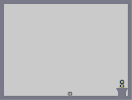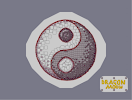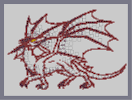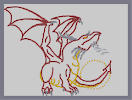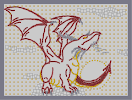The story of a small plant 3D Yin-Yang Nam dragon Afternoon Dragon Step 1 Afternoondragon Step02 Afternoondragon Step03

Pages: (17) [ 1 ] 2 3 4 5 6 7 8 9 10 11 12 13 14 15 16 17

### 2022

2022
Demo Data 2022:2022

### I'm flabbergasted.

This is simply astonishing.

### This is pretty amazing.

Probably some of the few things more amazing than this are the number of comments on this map (689, once this is submitted) and the number of ratings (1263, once I've added my five-ninja rating to the horde).

~BOB2

:)

### Just thought I'd add my voice to the masses...

5/5

Once you do this, you will appreciate it like I do. I don't think I can exaggerate how much I love this map and how much it blows my mind, which is why it kills me to say this. After watching this load 3 times, I have found that there are 2 ( STILL JUST A TINY AMOUNT!) mistakes.
1. The place where the closer wing comes out of the body looks wrong.
2. The length of the neck, and also the back of the neck, look awkward.
Still, an amazing map.
5/5 & 5aved

### lol, top rated

1. Afternoondragon Step04
2. N-Art
3. Tileset
4. Yahoozy
5. N-Art
6. Tileset
7. Stepself
8. N-Art
9. Stepself
10. wellsj

lame"

### Top rated was good

It gave us highlights much more efficiently than hot maps, active authors and featured maps do. Except the imagined discrepancy is probably down to the number of decent maps being made. I can't remember if there was a daily / weekly / monthly top rated or if it was just all-time.
timeless.

### origami_alligator sed

this shall not be featured... ;P

### Hasn't aged that well, IMO.

The comments on this map are amazing, however. There's a huge chunk of NUMA's history preserved within these comments.
Not popular enough imo

### Now I look like an idiot...

Oh well. :)
I miss browsing maps by rating. Bring it back, please.

### While you're featuring N-Arts

Go through some of mine ;)

Great art by DM

### Thar she blows,

holding up well to time too.

### oh also i was being sarcastic

apparently that didn't transfer well
Kinda ironic, it was featured right after my comment.

### best n art

nice tho i prefer levels you can play - still great n art
4/5 only because you cant play

Good feature.

### THIS IS GLORIOUS

WE REALLY NEED TOP RATED AND SORTING BY AUTHORS AGAIN; IT WOULD MAKE THIS WEBSITE VIBRANT

### Hmmm

Kinda agree with mahi. Though i would like some more maps that are fun to try and do well on. All we ever get are action maps/minejumpers which the reviewers deem to be original, this isnt a rant btw i would just prefer it that way :P
Also: yessssssss. I commented on very first page of this map. Some hipster shit right there.

### fantastic use of a feature

i got tired of seeing boring "action" maps, we should feature n-arts every week

2006?!

NOT THIS AGAIN!

### 3

if it was a cat it would be a 4
We had this many users on NUMA?

.

bye

### 6 years..

1200 ratings..

I remember this.. wow. I've been here for ages.